# Excel DAX - Overview

DAX stands for Data Analysis Expressions. DAX is a formula language and is a collection of functions, operators, and constants that can be used in a formula or expression to calculate and return one or more values. DAX is the formula language associated with the Data Model of Excel Power Pivot.

It is not a programming language, but is a formula language that allows the users to define custom calculations in calculated columns and calculated fields (also known as measures). DAX helps you create new information from the data that is already present in your Data Model. DAX formulas enable you to perform data modeling, data analysis, and use the results for reporting and decision making.

DAX includes some of the functions that are used in Excel formulas, but with modified functionality and additional functions that are designed to work with relational data and perform dynamic aggregation.

## Importance of DAX

The foundation of DAX is the Data Model that is the Power Pivot database in Excel. Data Model consists of tables between which relationships can be defined so as to combine the data from different sources. The data connections to the Data Model can be refreshed as and when the source data changes. Data Model makes the use of the Power Pivot xVelocity in-memory analytics engine (VertiPaq) that makes the data operations to be as quick as possible in addition to accommodating several thousands of rows of data. For more information on Data Model, refer to the tutorial – Power Pivot.

DAX in conjunction with Data Model enables several power features in Excel – Power Pivot, Power PivotTables, Power PivotCharts and Power View. You can use DAX to solve a number of basic calculations and data analysis problems.

DAX is also useful in Power BI to create a new Power BI Desktop file and import some data into it. Further, DAX formulas provide capabilities such as analyzing growth percentage across product categories and for different date ranges, calculating year-over-year growth compared to market trends and many others as well.

Learning how to create effective DAX formulas will help you get the most out of your data. When you get the information you need, you can begin to solve real business problems that affect your bottom line. This is the power in Power BI and DAX will help you get there.

## Prerequisites for this Tutorial

This tutorial is an extension to Excel Power Pivot tutorial, where you have learnt about the Power Pivot feature, Data Model, Relationships, Power PivotTables, Power Pivot Charts, etc. It would be a good idea to brush up on this tutorial before you delve into DAX as this tutorial is more on the DAX language wherein you write formulas for the analysis of data in the Data Model and report those results.

This tutorial also introduces DAX Functions that are like Excel Functions, but with some variations. A comparison of Excel Functions and DAX Functions is provided to help you distinguish both. Similarly, Excel formulas and DAX formulas are compared and the similarities and differences are discussed. A good understanding of these differences would help you in writing effective DAX formulas efficiently.

Knowledge of Excel Functions and Excel Formulas is not necessary for this tutorial, as DAX is entirely for the Data Model in the Power Pivot window. You will get into an Excel worksheet only to view the Power PivotTables, Power Pivot Charts and Power View visualizations that are based on Data Model. However, if you are an Excel professional with good amount of knowledge in Excel Functions and Formulas, better make a note of what is mentioned in the previous section and the details given in the course of this tutorial.

## Calculated Columns

Calculated columns are the columns that you can add to a table in the Data Model, by means of a DAX formula. You have already learnt about them in Excel Power Pivot tutorial, but you will learn in detail in the chapter – Calculated Columns as DAX is all about calculated columns, calculated fields, and DAX functions.

## Calculated Fields / Measures

You cannot change the values in the tables in the Data Model by editing. However, you can add calculated fields to a table that can be used in the Power PivotTables. The calculated fields are defined by giving a name and by defining a DAX formula. For details, refer to the chapter – Calculated Fields.

The calculated fields were named as measures in the Excel versions prior to Excel 2013. They are renamed back to measures in Excel 2016. In this tutorial, we will refer them as calculated fields. But, note that the terms - calculated fields and measures - are synonymous and refer to the same in all aspects.

You can edit a calculated field after it is defined and stored. You can change the DAX formula used in the definition or you can rename the calculated field. You will learn about this in the chapter – Editing a Calculated Field. You can delete a calculated field. Refer to the chapter – Deleting a Calculated Field.

## DAX Formulas

DAX formulas form the heart of the DAX language. You can create calculated fields and calculated columns by defining them with DAX formulas. You can write DAX formulas for the data analysis operations. DAX formulas do not refer to the individual cells or range of cells in the table, but refer to the tables and columns in the Data Model. A column in a table in the Data Model must contain the same data type.

DAX formulas contain the tables, columns, calculated columns, calculated fields, DAX operators, and DAX functions. Refer to the chapter – DAX Formulas to learn in detail.

## DAX Syntax

As is the case with any language, DAX, the formula language also has a syntax. Your DAX formulas should follow DAX syntax, or else, you will either get errors at design time or at run time or you will receive incorrect results.

You will learn the following in the chapter – DAX Syntax

• DAX naming requirements for Tables, Columns
• DAX operators
• DAX special values
• DAX data types
• DAX implicit data type conversions

## DAX Operators

DAX is a formula language and hence makes the use of the operators in defining the formulas. DAX has the following types of operators −

• DAX Arithmetic Operators
• DAX Comparison Operators
• DAX Text Concatenation Operator
• DAX Logical Operators

DAX operator precedence order is also defined and varies from Excel operator precedence order. Refer to the chapter – DAX Operators.

## DAX Standard Parameters

DAX Function syntax has certain requirements on parameters. This is because the DAX function arguments can be tables or columns or calculated fields or other DAX functions. Refer to the chapter - DAX Standard Parameters.

## DAX Functions

Excel 2013 has 246 DAX functions that you can use in DAX formulas. You will learn about these functions at the category level in the chapter – DAX Functions. However, for details on each DAX function syntax, parameters, usage and return values, you have to refer to our tutorial on – DAX Functions. The section names used for the description of each DAX function is given in the chapter – Understanding DAX Functions.

As DAX functions are required in writing the DAX formulas and the results of the DAX functions used depend on the context they are used, you might have to go back and forth between these two tutorials to get a grasp on DAX that you will use in Data Modeling with DAX and Power BI.

## DAX Special Functions

DAX has some functions that make DAX powerful. These DAX functions come under the categories – DAX time intelligence functions and DAX filter functions and require a special mention. You will learn about DAX time intelligence functions in the chapter – Understanding DAX Time Intelligence. You will learn about the usage of DAX filter functions in the chapter – DAX Filter Functions.

## DAX Evaluation Context

The results of a DAX formula can vary based on the context that is used for evaluation. DAX has two types of evaluation context – Row Context and Filter Context. Refer to the chapter - DAX Evaluation Context.

## DAX Formulas

DAX is a formula language and you have to get the most of it in writing the DAX formulas. Refer to the chapter - DAX Formulas to learn about the formula syntax and how to create them easily and correctly.

The results of the DAX formulas change whenever the data is refreshed and whenever the DAX formulas are recalculated. You have to understand the difference between data refresh and recalculation. Refer to the chapter - Updating the Results of DAX Formulas.

Data in the Data Model is expected and subjected to change from time to time. This is because the data is used for data analysis activities that require up-to-date data at any point of time. To understand the different ways of refreshing data, refer to the chapter - Updating Data in Data Model.

You will understand the different types of DAX formula recalculation in the chapter - Recalculating DAX Formulas.

DAX formula recalculations have to consider data dependencies and follow a specific order. Otherwise, you might get errors or erroneous results. Refer to the chapter - Troubleshooting DAX Formula Recalculation for details.

You will get an insight into some of the common DAX formula errors and you will learn how to fix those errors, in the chapter - DAX Formula Errors.

## DAX Scenarios

If you start learning a new language, the best way of getting acquainted to the language is by understanding where to use what. Similarly, DAX being a formula language meant for data analysis, you need to understand the various scenarios where it can be used.

Refer to the following chapters to get details on this.

# Excel DAX - Calculated Columns

A calculated column is a column that you add to an existing table in the Data Model of your workbook by means of a DAX formula that defines the column values. Instead of importing the values in the column, you create the calculated column.

You can use the calculated column in a PivotTable, PivotChart, Power PivotTable, Power PivotChart or Power View report just like any other table column.

## Understanding Calculated Columns

The DAX formula used to create a calculated column is like an Excel formula. However, in DAX formula, you cannot create different formulas for different rows in a table. The DAX formula is automatically applied to the entire column.

For example, you can create one calculated column to extract Year from the existing column – Date, with the DAX formula −

```= YEAR ([Date])
```

YEAR is a DAX function and Date is an existing column in the table. As seen, the table name is enclosed in brackets. You will learn more about this in the chapter – DAX Syntax.

When you add a column to a table with this DAX formula, the column values are computed as soon as you create the formula. A new column with the header CalculatedColumn1 filled with Year values will get created.

Column values are recalculated as necessary, such as when the underlying data is refreshed. You can create calculated columns based on existing columns, calculated fields (measures), and other calculated columns.

## Creating a Calculated Column

Consider the Data Model with the Olympics Results as shown in the following screenshot.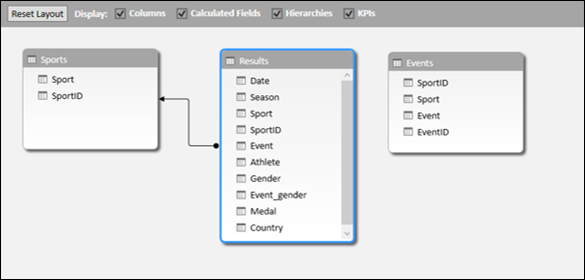• Click the Data View.
• Click the Results tab.

You will be viewing the Results table.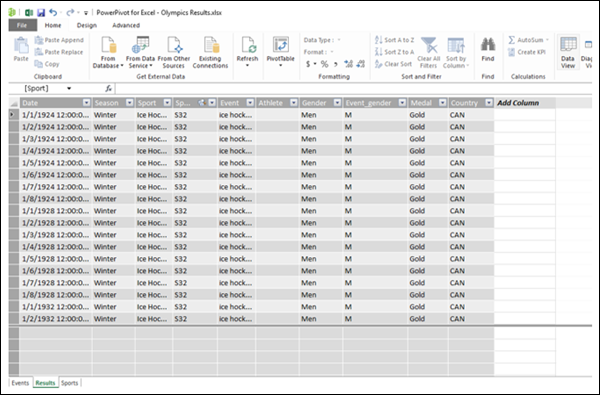As seen in the above screenshot, the rightmost column has the header – Add Column.

• Click the Design tab on the Ribbon.
• Click Add in the Columns group.The pointer will appear in the formula bar. That means you are adding a column with a DAX formula.

• Type =YEAR ([Date]) in the formula bar.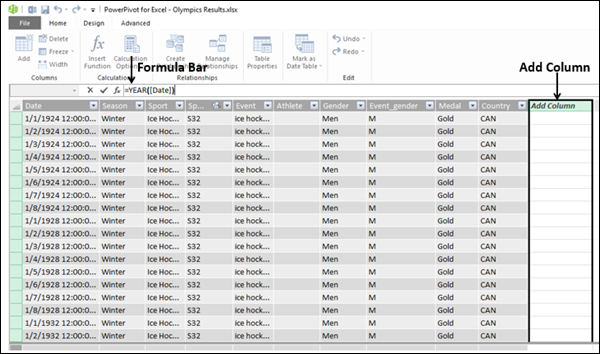As can be seen in the above screenshot, the rightmost column with the header – Add Column is highlighted.

• Press Enter.

It will take a while (few seconds) for the calculations to be done. Please wait.

The new calculated column will get inserted to the left of the rightmost Add Column.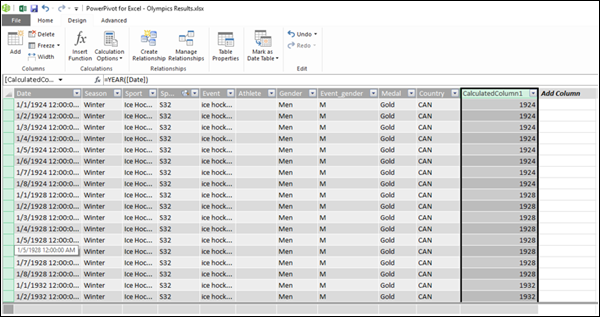As shown in the above screenshot, the newly inserted calculated column is highlighted. Values in the entire column appear as per the DAX formula used. The column header is CalculatedColumn1.

## Renaming the Calculated Column

To rename the calculated column to a meaningful name, do the following −

• Double-click on the column header. The column name will be highlighted.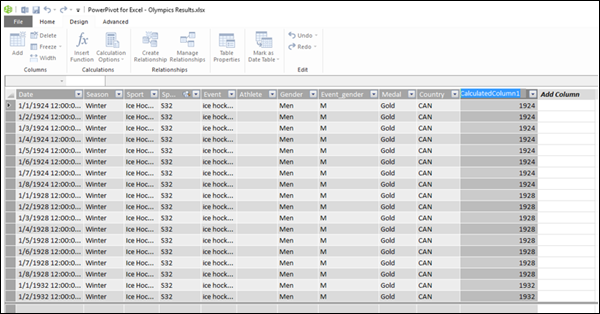• Select the column name.
• Type Year (the new name).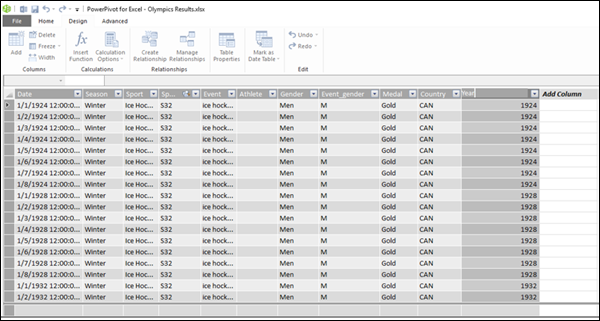As seen in the above screenshot, the name of the calculated column got changed.

You can also rename a calculated column by right-clicking on the column and then clicking on Rename in the dropdown list.

Just make sure that the new name does not conflict with an existing name in the table.

## Checking the Data Type of the Calculated Column

You can check the data type of the calculated column as follows −

• Click the Home tab on the Ribbon.
• Click the Data Type.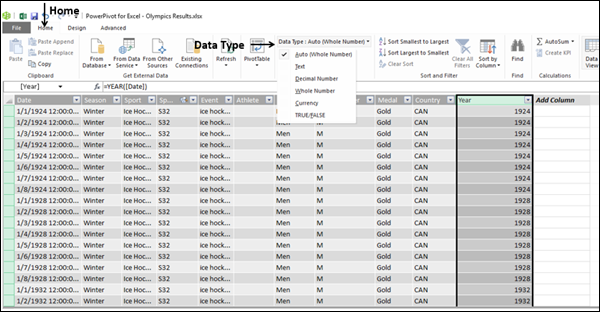As you can see in the above screenshot, the dropdown list has the possible data types for the columns. In this example, the default (Auto) data type, i.e. the Whole Number is selected.

## Errors in Calculated Columns

Errors can occur in the calculated columns for the following reasons −

• Changing or deleting relationships between the tables. This is because the formulas that use columns in those tables will become invalid.

• The formula contains a circular or self-referencing dependency.

## Performance Issues

As seen earlier in the example of Olympics results, the Results table has about 35000 rows of data. Hence, when you created a column with a DAX formula, it had calculated all the 35000+ values in the column at once, for which it took a little while. The Data Model and the tables are meant to handle millions of rows of data. Hence, it can affect the performance when the DAX formula has too many references. You can avoid the performance issues doing the following −

• If your DAX formula contains many complex dependencies, then create it in steps saving the results in new calculated columns, instead of creating a single big formula at once. This enables you to validate the results and assess the performance.

• Calculated columns need to be recalculated when data modifications occur. You can set the recalculation mode to manual, thus saving frequent recalculations. However, if any values in the calculated column are incorrect, the column will be grayed out, until you refresh and recalculate the data.

# Excel DAX - Calculated Fields / Measures

A calculated field in a table in a Data Model is the field obtained by a DAX formula. In earlier versions of Power Pivot, the calculated field was termed as a measure. In Excel 2013, it was renamed as a calculated field. However, it is renamed back to measure in Excel 2016. If you refer to any documentation, you can observe a mix up of these two terms. Note that the terms calculated field and measure are synonymous. In this tutorial, we use the term calculated field.

## Understanding Calculated Fields

A calculated field is a formula that is created specifically for use in a PivotTable (or PivotChart).

You can create a calculated field based on standard aggregation functions, such as COUNT or SUM, or by defining your own DAX formula.

Following is the difference between the calculated field and the calculated column −

• A calculated field can be used only in the VALUES area of a PivotTable.

• A calculated column with the calculated results can be used in ROWS, COLUMNS and FILTERS areas also.

### Saving Calculated Field

The calculated field will be saved with its source table in the Data Model. It appears in the Power PivotTable or Power PivotChart Fields list as a field in the table.

### Using Calculated Field

To use a calculated field, you have to select it from the Power PivotTable Fields list. The calculated field will get added to the VALUES area and the formula used for the calculated field will be evaluated. A result is created for each combination of row and column fields.

## Calculated Field – An Example

Consider the following Data Model for Olympics data −As seen in the above screenshot, the Results table has a field Medal that contains the values – Gold, Silver, or Bronze for each of the rows containing Sport – Event - Country – Date combination. Suppose you want medal count for each country, then you can create a calculated field Medal Count with the following DAX formula −

```Medal Count := COUNTA([Medal])
```

## Creating a Calculated Field in a Table

To create the calculated field Medal Count in the Results table, do the following −

• Click the cell in the calculation area below the Medal column in the Results table. The cell will be highlighted.

• Type Medal Count:=COUNTA([Medal]) in the formula bar.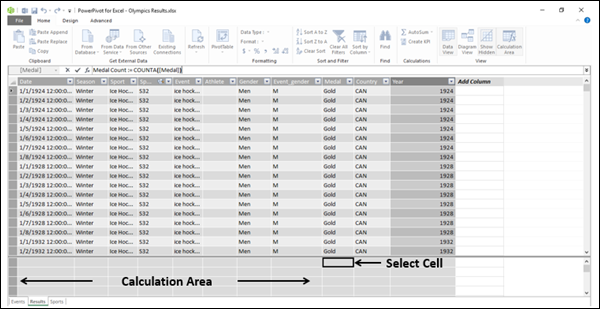Press Enter.As seen in the above screenshot, the calculated field appears in the selected cell, showing the value as 34,094. This number is the total number of rows in the Results table. Hence, it does not make much sense at the first look. As discussed earlier, the real use of a calculated field can be seen only by adding it to a Power PivotTable or a Power PivotChart.

## Using the Calculated Field in a Power PivotTable

To use the calculated field to count the number of medals for each country, do the following −

• Click the PivotTable on the Ribbon in the Power Pivot window.
• Click the PivotTable in the dropdown list.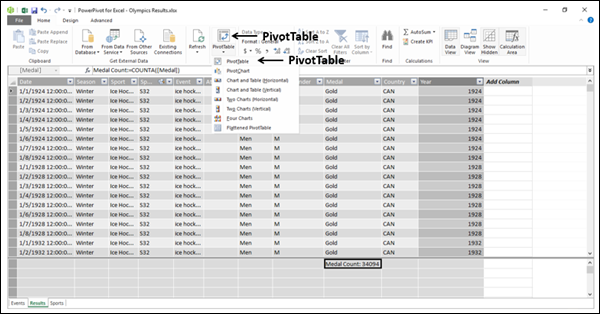Create PivotTable dialog box appears.

• Click the Existing Worksheet.
• Select where you want to place the PivotTable.

An empty PivotTable will get created.

• Click the Results table in the PivotTable Fields list.
• Click the fields – Country and Medal count.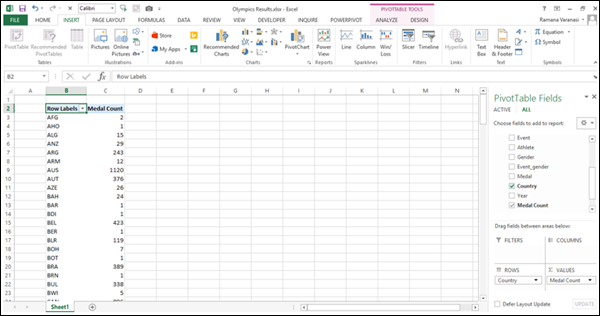As you can observe, Medal Count is added to VALUES area and Country is added to ROWS area. The PivotTable is created with the field Country values appearing in the rows. And for each row, the Medal Count value is calculated and displayed. That is the way, the calculated field evaluates the DAX formula used and displays the values.

• Add the field Sport from the Results table to ROWS area.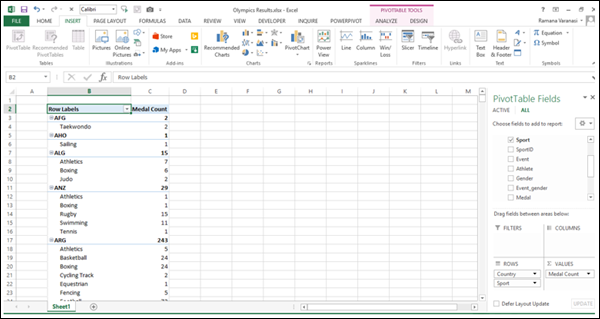As you can see in the above screenshot, Medal Count is calculated for each Country - Sport-wise and a Subtotal for the Country itself.

This is how DAX supplements the Power features.

## Types of Calculated Fields

There are two types of Calculated Fields – Implicit and Explicit.

• An implicit calculated field is created in the Power PivotTable Fields list pane.

• An explicit calculated field is created either in the table in the Power Pivot window, or from the PowerPivot Ribbon in the Excel window.

## Creating an Implicit Calculated Field

An implicit calculated field can be created in two ways, both in the Power PivotTable Fields pane.

### Creating an Implicit Calculated Field in the PivotTable Fields List

You can create the Count of Medal Field from the Medal field in the PivotTable Fields list as follows −

• Deselect the field Medal Count.
• Right-click on the field Medal.
• Click Add to Values in the dropdown list.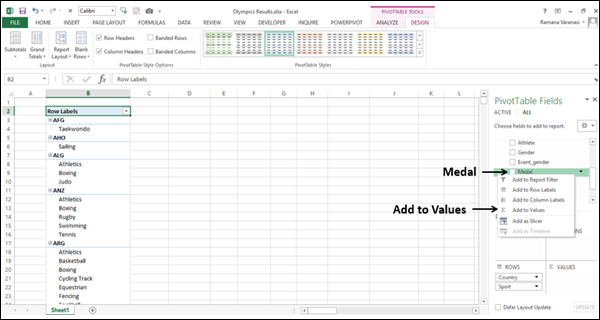Count of Medal appears in the Values area. Count of Medal column will be added to the PivotTable.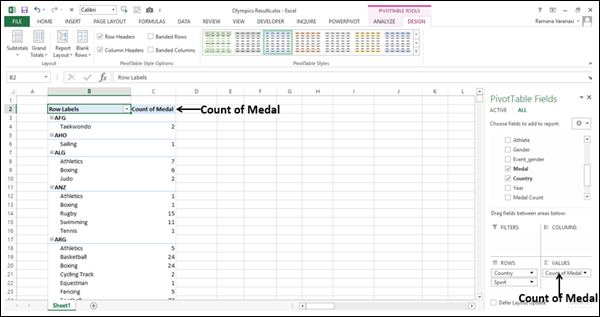### Creating an Implicit Calculated Field in the VALUES Area

You can create an implicit calculated field - % of Parent Row in the Values area to express the Medal count of each sport that a country has won as a percentage of the total number of Medals won by that Country.

• Click the down arrow in the Count of Medal box in VALUES area.
• Click the Value Field Settings in the dropdown list.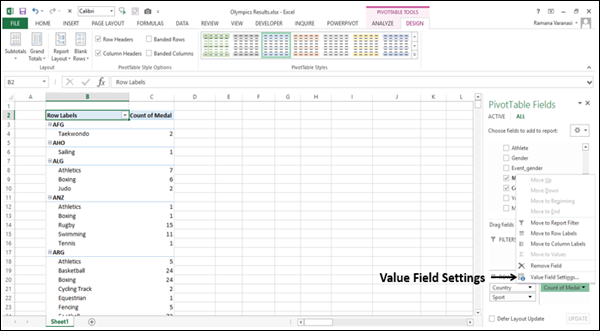Value Field Settings dialog box appears.

• Type % Medals in the Custom Name box.
• Click the Show Values As tab.
• Click the box under Show values as.
• Click the % of Parent Row Total.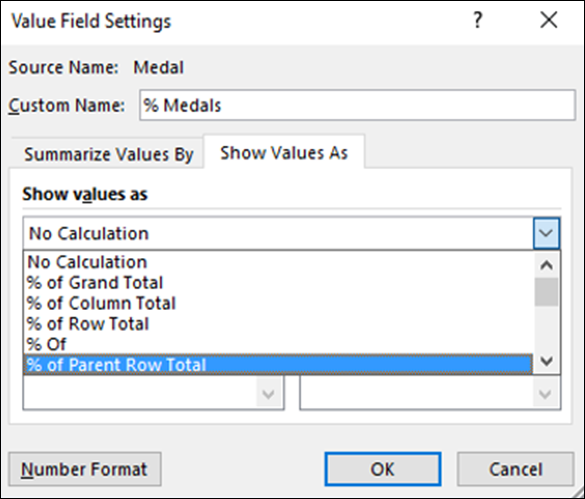• Click the Number Format button.

Format Cells dialog box appears.

• Click Percentage.
• Type 0 in decimal places.
• Click OK.
• Click OK in the Value Field Settings dialog box.
• Select Do Not Show Subtotals.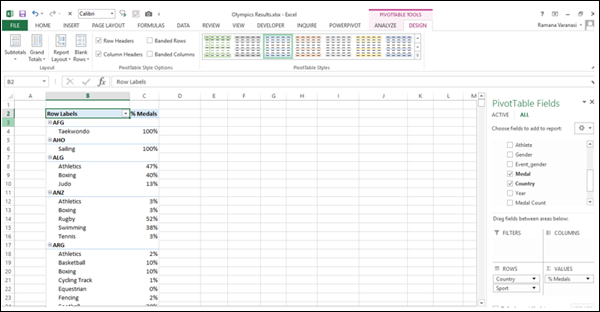You created another implicit calculated field % Medals and as you can observe, for each Country, the percentage of Medals Sport-wise are displayed.

## Drawbacks of an Implicit Calculated Field

Implicit calculated fields are easy to create. In fact, you have been creating them even in Excel PivotTables and Pivot Charts. But, they have the following drawbacks −

• They are volatile. That means, if you deselect the field you used for calculated field, it will be removed. If you want to display it again, you have to once again create it.

• Their scope is limited to the PivotTable or PivotChart in which they are created. If you create another PivotTable in another worksheet, you have to create the calculated field again.

On the other hand, explicit calculated fields will get saved with the table and will be available whenever you select that table.

## Creating an Explicit Calculated Field

You can create an explicit calculated field in two ways −

• In the calculation area in a Table in the Data Model. You have already learnt this in the section – Creating Calculated Field in a Table.

• From PowerPivot Ribbon in the Excel table. You will learn this way of creating an explicit calculated field in the next section.

### Creating an Explicit Calculated Field from PowerPivot Ribbon

To create an explicit calculated field from PowerPivot Ribbon, do the following −

• Click the POWERPIVOT tab on the Ribbon in your workbook.
• Click the Calculated Fields in the Calculations area.
• Click the New Calculated Field in the dropdown list.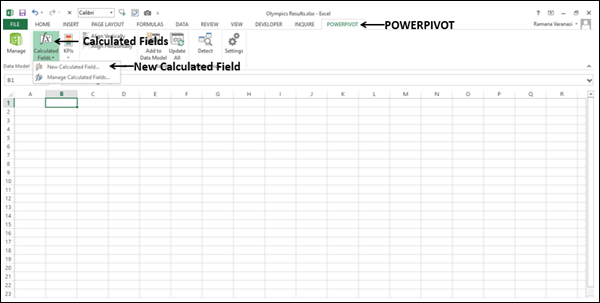Calculated Field dialog box appears.

• Fill in the required information as shown in the following screenshot.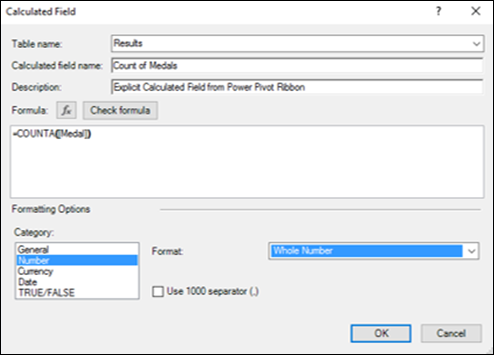• Click the Check formula button.
• Click OK only if there are no errors in the formula.

As you can observe, you can define the category and format of the calculated field in this dialog box. Further, you can use the IntelliSense feature to understand the usage of the functions and to use the AutoComplete feature to easily complete the names of the functions, tables, and columns. For details on IntelliSense feature, refer to the chapter – DAX Formulas.

This is a recommended way to create explicit calculated fields.

# Excel DAX - Editing a Calculated Field

You can edit a calculated field to modify it. But, before you edit a calculated field, you should know where it is stored. That means, in which table the calculated field is stored. This holds both for implicit and explicit calculated fields. A calculated field can be associated with only one table in a Data Model.

## Finding Calculated Fields

To find the calculated fields in the Data Model, do the following −

• Click the Advanced tab on the Ribbon in the Power Pivot window.
• Click the Show Implicit Calculated Fields.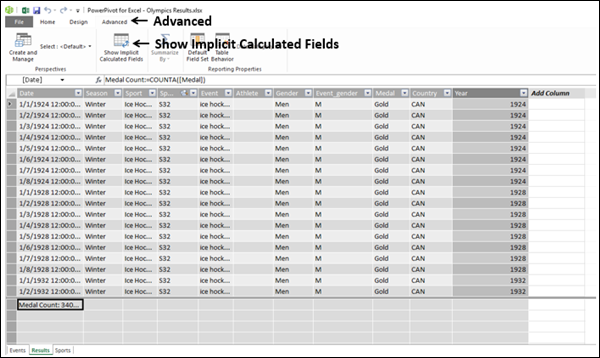• Click the Diagram View.As you can see in the above screenshot, Show Implicit Calculated Fields is highlighted on the Ribbon. If it is not highlighted, click it again.

You can also observe that there are 4 checkboxes – Columns, Calculated Fields, Hierarchies, and KPIs. By default, all the 4 are selected.

• Uncheck the boxes - Columns, Hierarchies and KPIs.

This will leave only Calculated Fields box checked.As seen in the above screenshot, only the Results table has fields displayed. The other two tables are blank. This shows that only the Results table has calculated fields. You can also observe that the implicit calculated fields have an icon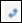displayed, whereas the explicit calculated field – Medal Count does not have that icon.

## Viewing Calculated Fields in the Table

You can view the calculated fields in the Table as follows −

• Click the calculated field.
• Right-click and select Go To in the dropdown list.The table will appear in Data View.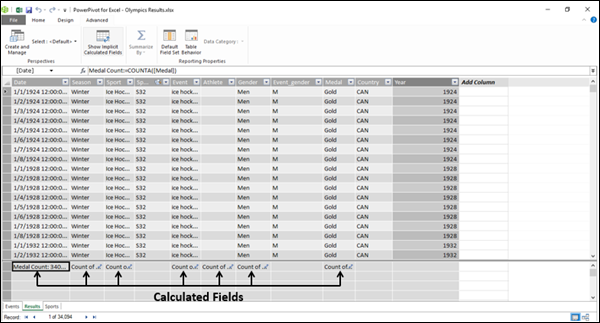As seen in the above screenshot, the calculated fields appear in the calculation area of the table.

## Changing a Calculated Field in the Table

You can change the formula used for a calculated field in the table.

• Click the calculated field in the table in data view of the Data Model.
• Select the formula in the formula bar – to the right side of :=.

The formula will get highlighted.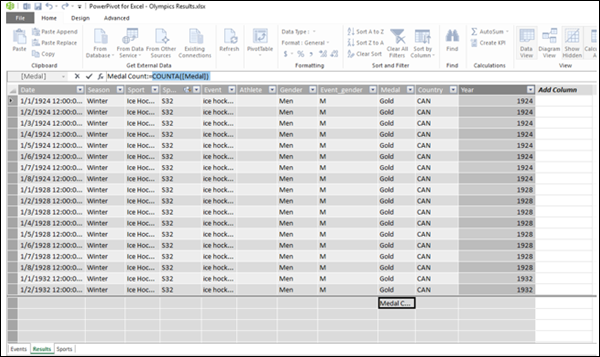• Type the new formula.
• Press Enter.

## Renaming a Calculated Field in the Data Model

You can change the name of a calculated field in the Data Table either in Data View or Diagram View.

### Renaming a Calculated Field in the Data View

• Click the calculated field in the table in data view of the Data Model.
• Select the calculated field name in the formula bar – to the left side of :=.

The calculated field name will get highlighted.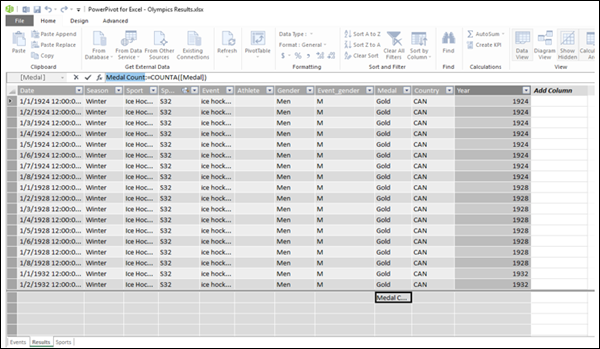• Type the new name for the calculated field.
• Press Enter.

### Renaming a Calculated Field in the Diagram View

• Right-click the calculated field name in the table in the diagram view.
• Click Rename in the dropdown list.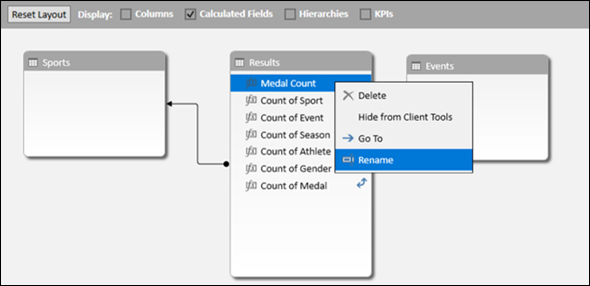The name will get into editing mode. Type the new name for the calculated field.

## Viewing Calculated Fields in the Excel Window

You can view the calculated fields in the Excel window as follows −

• Click the POWERPIVOT tab on the Ribbon.
• Click Calculated Fields in the Calculations group.
• Click Manage Calculated Fields in the dropdown list.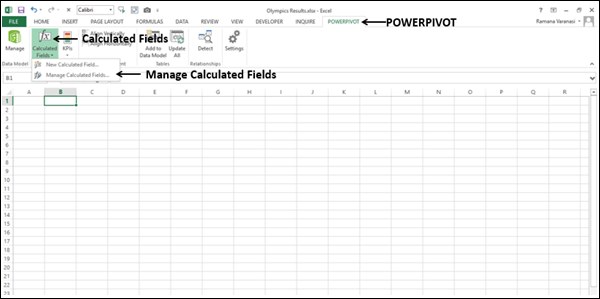Manage Calculated Fields dialog box appears. The names of the explicit calculated fields in the Data Model appear in the dialog box.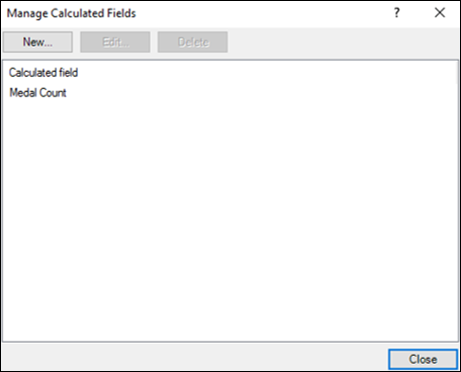## Changing a Calculated Field in the Manage Calculated Fields

You can change a calculated field in the Manage Calculated Fields dialog box.

• Click the Medal Count.
• Click the Edit button.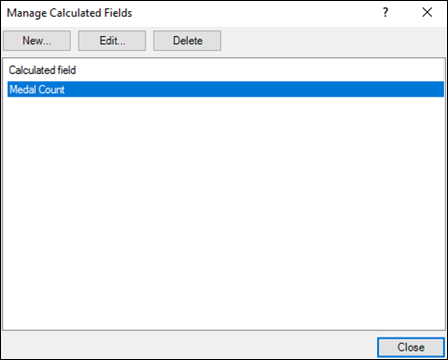Calculated Field dialog box appears.

• Select the formula to the right of = in the formula box.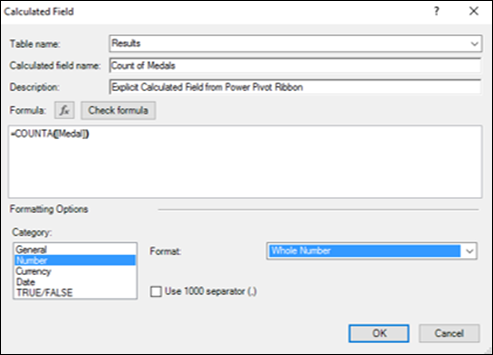• Type the new Formula.
• Click OK.
• Click Close in the Manage Calculated Fields dialog box.

## Renaming a Calculated Field in the Manage Calculated Fields

You can rename a calculated field in the Manage Calculated Fields dialog box.

• Click the Medal Count.
• Click the Edit button.

Calculated Field dialog box appears.

• Select the name in the calculated field name box.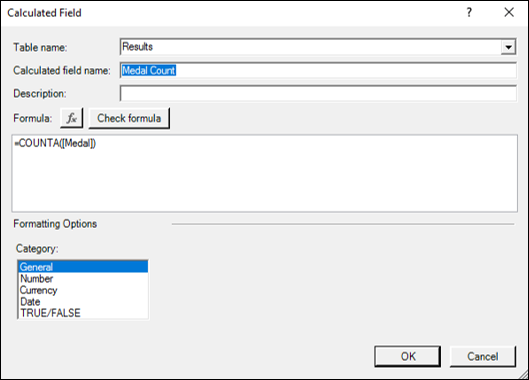• Type the new name for the calculated field.
• Click OK.
• Click Close in the Manage Calculated Fields dialog box.

## Moving a Calculated Field in the Data Model

You can move a calculated field within the calculation area of the table in which it is created. But, it cannot be moved to another table.

• Right-click the calculated field.
• Click Cut.
• Move the pointer to a different place in the calculation area of the same table.
• Click Paste.

Note − It does not really matter where the calculated field is within the calculation area of the table because the data references in the DAX formula of the calculated field are by the column names and are stated explicitly.

# Excel DAX - Deleting a Calculated Field

You can delete both explicit and implicit calculated fields. There are several ways of doing so, which you will learn in this chapter.

However, you need to remember the following points before deleting a calculated field −

• An explicit calculated field can be used in more than one PivotTable and/or PivotChart. Hence, you need to make sure that deleting the explicit calculated field does not affect any of the reports that you have already generated.

• An explicit calculated field can be used in the calculations of other explicit calculated fields. Hence, you need to make sure that the explicit calculated field is not used in any of the calculations of other explicit calculated fields.

• An implicit calculated field is limited to the PivotTable or PivotChart where it is used. Hence, before deleting an implicit calculated field, it is just sufficient to make sure that it can be deleted from the corresponding PivotTable or PivotChart.

• Creating an implicit calculated field is simpler than creating an explicit calculated field. Hence, more caution is required before deleting an explicit calculated field.

• You cannot create an explicit calculated field, if the name conflicts with the name of an implicit calculated field. Hence, you might have to delete that implicit calculated field before creating the explicit calculated field.

## Deleting an Explicit Calculated Field in the Data Model

You can delete an explicit calculated field either in the data view or the diagram view in the Data Model.

### Deleting an Explicit Calculated Field in the Data View

• Locate the calculated field in the calculations area in the Data View.
• Right-click the calculated field.
• Click Delete in the dropdown list.Message appears for delete confirmation.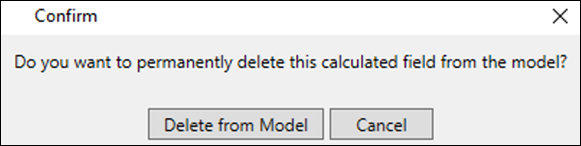Click Delete from Model. The explicit calculated field will get deleted.

### Deleting an Explicit Calculated Field in the Diagram View

• Locate the calculated field in the data table in the Diagram View.
• Right-click the calculated field name.
• Click Delete in the dropdown list.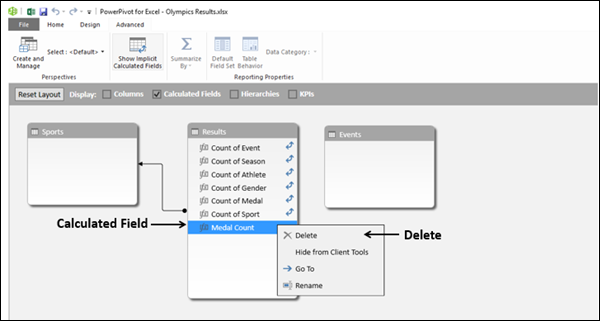Message appears for delete confirmation.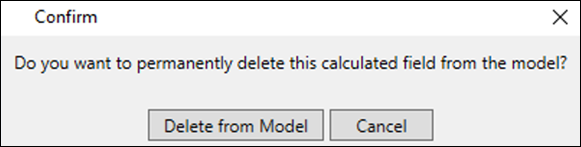Click Delete from Model. The explicit calculated field will get deleted and it will not be seen in the fields list of the data table.

### Deleting an Explicit Calculated Field in the Excel Window

You can delete an explicit calculated field from the Excel window as follows −

• Click the POWERPIVOT tab on the Ribbon.
• Click Calculated Field in the Calculations group.
• Click Manage Calculated Fields in the dropdown list.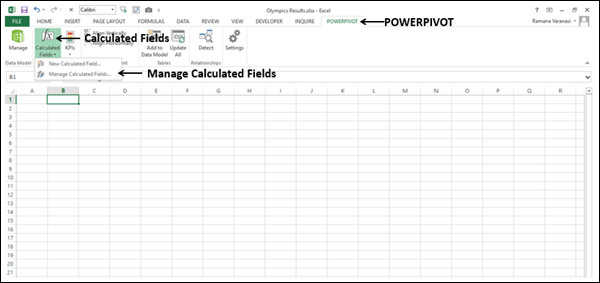Manage Calculated Fields dialog box appears.

• Click the explicit calculated field name.
• Click the Delete button.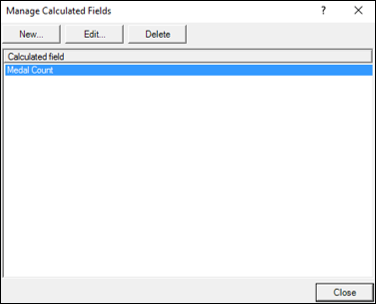Confirmation message for deletion appears.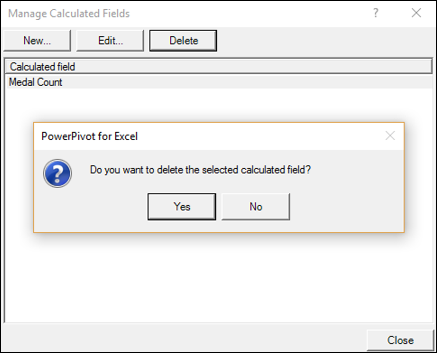• Click Yes. Information message that the Data Model is changed appears at the top.
• Click the Close button in the dialog box.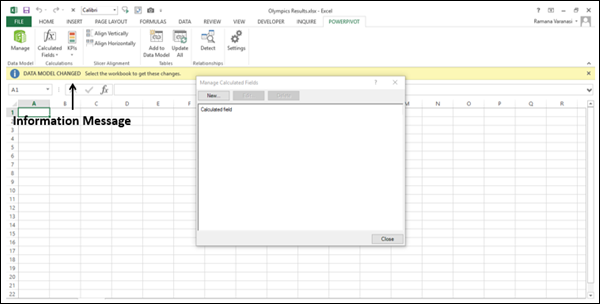The explicit calculated field will get deleted and it will not be seen in the PivotTable/PivotChart Fields list in the workbook.

## Deleting an Implicit Calculated Field

You can delete an implicit calculated field either in the data view or the diagram view in the Data Model.

### Deleting an Implicit Calculated Field in the Data View

• Locate the calculated field in the calculations area in the Data View.
• Right-click the calculated field.
• Click Delete in the dropdown list.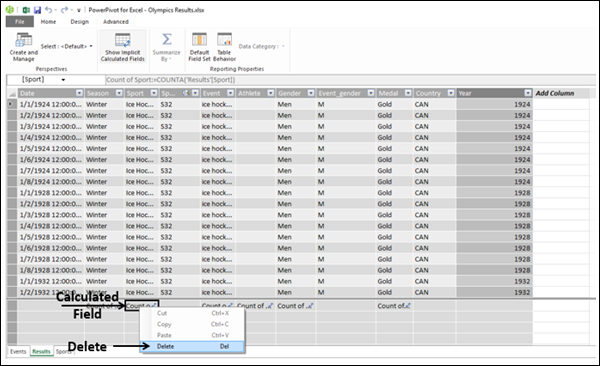Message appears for delete confirmation.• Click Delete from Model. The implicit calculated field will get deleted.

### Deleting an Implicit Calculated Field in the Diagram View

• Locate the calculated field in the data table in the Diagram View.
• Right-click the calculated field name.
• Click Delete in the dropdown list.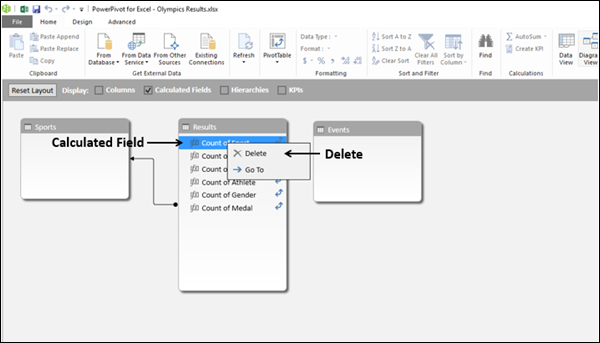Message appears for delete confirmation.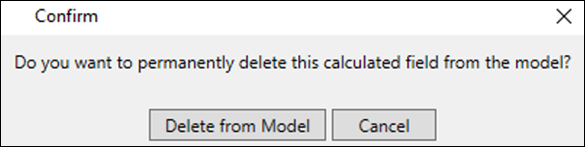Click Delete from Model. The implicit calculated field will get deleted and it will not be seen in the fields list of the data table.

# Excel DAX - Syntax

As discussed earlier, DAX is a formula language comprising of operators, values, functions, and formulas. In this chapter, you will learn about DAX Syntax.

DAX Syntax can be categorized as −

Before you proceed to learning DAX Syntax, you have to understand the difference between Excel formulas and DAX formulas.

## Differences between Excel Formulas and DAX Formulas

DAX formulas are similar to the Excel formulas and you can type them in the formula bar. However, there are some vital differences between the two.

Excel Formula DAX Formula

Excel formulas are typed in the formula bar in the Excel window.

DAX formulas are typed in the formula bar in the Power Pivot window.

In Excel formulas, you can reference individual cells or arrays for data.

In DAX formulas, you can reference only complete tables or columns of data, i.e. references can be only to tables and fields in the tables.

However, if at all you have to perform a calculation only on a part of the column data, you can do so with the DAX functions that filter and provide the required unique data values for calculation.

Excel formulas support certain data types.

DAX provides more data types than Excel does. Hence, DAX formulas can use the additional data types also.

Excel does not support any implicit data conversions.

DAX performs implicit data type conversions during calculations.

# Excel DAX - Operators

DAX is a formula language comprising of functions, operators, and values that can be used in a formula or expression, to calculate and return one or more values.

You can use DAX operators to compare values, perform arithmetic calculations, and concatenate strings. In this chapter, you will learn about DAX operators and how to use them.

## Types of DAX Operators

DAX supports the following types of operators −

## DAX Operator Precedence Order

You can have a DAX formula with many DAX operators combining several values or expressions. In such a case, the final result will depend on the order in which the operations are performed. DAX provides you with the default operator precedence order and also ways of overriding the default precedence order.

DAX default operator precedence is listed in the following table.

Precedence Order Operator(s) Operation
1 ^ Exponentiation
2 Sign
3 * and / Multiplication and Division
4 ! NOT
5 + and – Addition and Subtraction
6 & Concatenation
7 =, <, >, <=, >= and <> Equal to, Less than, Greater than, Less than or equal to, Greater than or equal to and Not equal to

## DAX Expression Syntax

You need to first understand the DAX expression syntax and how the expression evaluation is done with the operands and operators.

• All expressions always begin with an equal sign (=). The equal sign indicates that the succeeding characters constitute an expression.

• To the right of the equal sign, you will have the operands connected by the DAX operators. For example, = 5 + 4 > 5.

= 5 * 6 - 3.

• Expressions are always read from left to right, and the calculations are done in that sequence, based on the DAX operator precedence given in the previous section.

• If the DAX operators have equal precedence value, they are evaluated from the left to right. For example, =5*6/10. Both * and / have same the precedent order. Hence, the expression is evaluated as 30/10 = 3.

• If the DAX operators in the expression have different precedence values, then they are evaluated in the precedence order from the left to right.

• = 5 + 4 > 7. Default precedence is + first and > next. Hence, the expression is calculated from the left to right. - 5 + 4 is calculated first resulting in 9 and then 9 > 5 is evaluated that results in TRUE.

• = 5 * 6 - 3. Default precedence is * first and - next. Hence, the expression is calculated from the left to right. - 5 * 6 is calculated first resulting in 30 and then 30 - 3 is calculated that results in 27.

• = 2 * 5 - 6 * 3. Default precedence is * first, * next and then -. Hence, the expression evaluates as 10 – 18 and then as -8. Note, that it is not 10 - 6 resulting in 4 and then 4*3 that is 12.

## Using Parentheses to Control DAX Calculation Order

You can change the DAX default operator precedence order by using parentheses, grouping the operands and the operators to control the calculation sequence.

For example, = 5 * 6 - 3 evaluates to 27 with the DAX default operator precedence order. If you use parenthesis to group the operands and operators as = 5 * (6 - 3), then 6 - 3 is calculated first resulting in 3 and then 5 * 3 is calculated which results in 15.

= 2 * 5 - 6 * 3 evaluates to -8 with the DAX default operator precedence order. If you use parenthesis to group the operands and operators as = 2 * (5 - 6) * 3, then 5 - 6 is calculated first resulting in -1 and then 2 * (-1) * 3 is calculated which results in -6.

As you can see, with the same operands and operators, different results are possible by the way you group them. Hence, when you use the DAX operators in the DAX formulas, you should pay attention to how the computation sequence is to be.

## Differences Between Excel and DAX

Though DAX has similarities with Excel formulas, there are certain significant differences between the two.

• DAX is more powerful than Excel because of its underlying memory resident computation engine.

• DAX supports more data types than Excel.

• DAX provides additional advanced features of a relational database, Data Model, including richer support for date and time types.

In some cases, the results of calculations or the behavior of functions in DAX may not be the same as in Excel. This is due to the differences in the following −

• Data type casting
• Data types

### Difference in Data Type Casting

In DAX, when you have an expression =value1 operator value2, the two operands value1 and value2 should be of the same data type. If the data types are different, DAX will convert them first to a common data type implicitly. Refer to the chapter – DAX Syntax for details.

For example, you have to compare two operands of different data types, say a number resulting from a formula, such as =[Amount] * 0.08 and an integer. The first number can be a decimal number with many decimal places, whereas the second number is an integer. Then DAX handles it as follows −

• First, DAX will convert both the operands to real numbers using the largest numeric format that can store both kinds of numbers.

• Next, DAX will compare the two real numbers.

In contrast, Excel tries to compare values of different data types without first coercing them to a common data type. For this reason, you might find different results in DAX and in Excel for the same comparison expression.

### Difference in Data Types

The operator precedence order in DAX and Excel is the same. However, the operator percent (%) and data ranges that Excel supports are not supported by DAX. Moreover, DAX supports table as a data type, which is not the case in Excel.

Further, in Excel formulas, you can refer to a single cell, or an array or a range of cells. In DAX formulas, you cannot refer to any of these. The DAX formula references to data should be by tables, columns, calculated fields, and calculated columns.

If you copy formulas from Excel and paste them in DAX, ensure the correctness of the DAX formula as DAX syntax is different from Excel formula syntax. Also, even if a function has the same name in DAX and Excel, its parameters might be different and the result of the function can also be different.

# Excel DAX - Standard Parameters

DAX has standard parameter names to facilitate the usage and understanding of the DAX functions. Further, you can use certain prefixes to the parameter names. If the prefix is clear enough, you can use the prefix itself as the parameter name.

## Standard Parameter Names

Following are the DAX standard parameter names −

Sr.No. Parameter Name & Description
1

expression

Any DAX expression that returns a single scalar value, where the expression is to be evaluated multiple times (for each row/context).

2

value

Any DAX expression that returns a single scalar value where the expression is to be evaluated exactly once before all other operations.

3

table

Any DAX expression that returns a table of data.

4

tableName

The name of an existing table using standard DAX syntax. It cannot be an expression.

5

columnName

The name of an existing column using standard DAX syntax, usually fully qualified. It cannot be an expression.

6

name

A string constant that will be used to provide the name of a new object.

7

order

An enumeration used to determine the sort order.

8

ties

An enumeration used to determine the handling of tie values.

9

type

An enumeration used to determine the data type for PathItem and PathItemReverse.

## Prefixing Parameter Names

You can qualify a parameter name with a prefix −

• The prefix should be descriptive of how the argument is used.

• The prefix should be in such a way that ambiguous reading of the parameter is avoided.

For example,

• Result_ColumnName − Refers to an existing column used to get the result values in the DAX LOOKUPVALUE () function.

• Search_ColumnName − Refers to an existing column used to search for a value in the DAX LOOKUPVALUE () function.

## Using Only the Prefix as a Parameter

You can omit the parameter name and use only the prefix, if the prefix is clear enough to describe the parameter. Omitting the parameter name and using only the prefix can sometimes help in avoiding the clutter in reading.

For example, Consider DATE (Year_value, Month_value, Day_value). You can omit the parameter name – value, that is repeated thrice and write it as DATE (Year, Month, Day). As you can observe, by using only the prefixes, the function is more readable.

However, sometimes the parameter name and the prefix have to be present for clarity.

For example, Consider Year_columnName. The parameter name is ColumnName and the prefix is Year. Both are required to make the user understand that the parameter requires a reference to the existing column of years.

# Excel DAX - Functions

Most of the DAX functions have the same names and functionality as that of Excel functions. However, DAX functions have been modified to use DAX data types and to work with tables and columns.

DAX has some additional functions that you will not find in Excel. These DAX functions are provided for specific purposes such as lookups based on relationships associated with the relational database aspects of the Data Model, the ability to iterate over a table to perform recursive calculations, to perform dynamic aggregation, and for calculations utilizing time intelligence.

In this chapter, you will learn about the functions supported in the DAX language. For more information on the usage of these DAX functions, refer to the tutorial – DAX Functions in this tutorials library.

## What is a DAX Function?

A DAX function is an in-built function provided in the DAX language to enable you to perform various actions on the data in the tables in your Data Model. As discussed earlier, DAX is used for data analysis and business intelligence purposes that require support to extract, assimilate, and derive insights from the data. The DAX functions that are based on the Data Model provide you with these utilities that make your job simpler, once you get a grasp on the DAX language and the usage of the DAX functions.

## Excel Functions vs. DAX Functions

There are certain similarities between Excel functions that you are aware of and the DAX functions. However, there are certain differences too. You need to get a clarity on these, so that you can avoid making mistakes in the usage of DAX functions and in writing DAX formulas that include DAX functions.

### Similarities between Excel Functions and DAX Functions

• Many DAX functions have the same name and the same general behavior as Excel functions.

• DAX has lookup functions that are similar to the array and vector lookup functions in Excel.

### Differences between Excel Functions and DAX Functions

• DAX functions have been modified to take different types of inputs and some of the DAX functions might return a different data type. Hence, you need to understand the usage of these functions separately though they have the same name. In this tutorial, you will find every DAX function prefixed with DAX so as to avoid confusion with the Excel functions.

• You cannot use DAX functions in an Excel formula or use Excel formulas/functions in DAX, without the required modifications.

• Excel functions take a cell reference or a range of cells as reference. DAX functions never take a cell reference or a range of cells as reference, but instead take a column or table as reference.

• Excel date and time functions return an integer that represents a date as a serial number. DAX date and time functions return a datetime data type that is in DAX but not in Excel.

• Excel has no functions that return a table, but some functions can work with arrays. Many of the DAX functions can easily reference complete tables and columns to perform calculations and return a table or a column of values. This ability of DAX adds power to the Power Pivot, Power View and Power BI, where DAX is used.

• DAX lookup functions require that a relationship is established between the tables.

• Excel supports variant data type in a column of data, i.e. you can have data of different data types in a column. Whereas, DAX expects the data in a column of a table to be always of the same data type. If the data is not of the same data type, DAX changes the entire column to the data type that best accommodates all the values in the column. However, if the data is imported and this issue arises, DAX can flag an error.

To learn about DAX data types and data type casting, refer to the chapter – DAX Syntax Reference.

## Types of DAX Functions

DAX supports the following types of functions.

• DAX Table Valued Functions
• DAX Filter Functions
• DAX Aggregation Functions
• DAX Time Intelligence Functions
• DAX Date and Time Functions
• DAX Information Functions
• DAX Logical Functions
• DAX Math and Trig Functions
• DAX Other Functions
• DAX Parent and Child Functions
• DAX Statistical Functions
• DAX Text Functions

In this section, you will learn about DAX functions at the functions category level. For details on the DAX Function Syntax and what the DAX function returns and does - refer to the DAX Functions tutorial in this tutorials library.

DAX time intelligence functions and DAX filter functions are powerful and require a special mention. Refer to the chapters - Understanding DAX Time Intelligence and DAX Filter Functions for details.

### DAX Table Valued Functions

Many DAX functions take tables as input or output tables or do both. These DAX functions are called DAX table valued functions. Because a table can have a single column, DAX table valued functions also take single columns as inputs. You have the following types of DAX table valued functions −

• DAX Aggregation functions
• DAX Filter functions
• DAX Time intelligence functions

Understanding DAX table valued functions helps you in writing DAX formulas effectively.

### DAX Aggregation Functions

DAX Aggregation functions aggregate any expression over the rows of a table and are useful in calculations.

Following are some DAX Aggregation functions −

• ADDCOLUMNS (<table>, <name>, <expression>, [<name>, <expression>] …)

• AVERAGE (<column>)

• AVERAGEA (<column>)

• AVERAGEX (<table>, <expression>)

• COUNT (<column>)

• COUNTA (<column>)

• COUNTAX (<table>, <expression>)

• COUNTBLANK (<column>)

• COUNTROWS (<table>)

• COUNTX (<table>, <expression>)

• CROSSJOIN (<table1>, <table2>, [<table3>] …)

• DISTINCTCOUNT (<column>)

• GENERATE (<table1>, <table2>)

• GENERATEALL (<table1>, <table2>)

• MAX (<column>)

• MAXA (<column>)

• MAXX (<table>, <expression>)

• MIN (<column>)

• MINA (<column>)

• MINX (<table>, <expression>)

• PRODUCT (<column>)

• PRODUCTX (<table>, <expression>)

• ROW (<name>, <expression>, [<name>, <expression>] …)

• SELECTCOLUMNS (<table>, <name>, <scalar_expression>,

• [<name>, <scalar_expression>] …)

• SUM (<column>)

• SUMMARIZE (<table>, <groupBy_columnName>, [<groupBy_columnName>] …, [<name>, <expression>] …)

• SUMX (<table>, <expression>)

• TOPN (<n_value>, <table>, <orderBy_expression>, [<order>], [<orderBy_expression>, [<order>]] …)

### DAX Filter Functions

DAX Filter functions return a column, a table, or values related to the current row. You can use DAX Filter functions to return specific data types, look up values in related tables, and filter by related values. DAX Lookup functions work by using tables and relationships between them. DAX Filter functions enable you to manipulate the data context to create dynamic calculations.

Following are some DAX Filter functions −

• ADDMISSINGITEMS(<showAllColumn>, [<showAllColumn>] …, <table>, <groupingColumn>, [<groupingColumn>] … [filterTable] …)

• ALL( {<table> | <column>, [<column>], [<column>] …} )

• ALLEXCEPT(<table>, <column>, [<column>] …)

• ALLNOBLANKROW(<table>|<column>)

• ALLSELECTED([<tableName> | <columnName>])

• CALCULATE (<expression>, <filter1>, <filter2>…)

• CALCULATETABLE (<expression>, <filter1>, <filter2>…)

• CROSSFILTER (<columnName1>, <columnName2>, <direction>)

• DISTINCT (<column>)

• EARLIER(<column>, <number>)

• EARLIEST(<column>)

• FILTER(<table>,<filter>)

• FILTERS(<columnName>)

• HASONEFILTER(<columnName>)

• HASONEVALUE(<columnName>)

• ISCROSSFILTERED (<columnName>)

• ISFILTERED (<columnName>)

• KEEPFILTERS (<expression>)

• RELATED(<column>)

• RELATEDTABLE(<tableName>)

• SUBSTITUTEWITHINDEX (<table>, <indexColumnName>, <indexColumnsTable>, <orderBy_expression>, [<order>], [<orderBy_expression>, [<order>]] …])

• USERELATIONSHIP(<columnName1>,<columnName2>)

• VALUES(<TableNameOrColumnName>)

### DAX Time Intelligence Functions

DAX Time Intelligence functions return a table of dates or use a table of dates to calculate an aggregation. These DAX functions help you create calculations that support the needs of Business Intelligence analysis by enabling you to manipulate data using time periods, including days, months, quarters and years.

Following are some DAX Time Intelligence functions −

• CLOSINGBALANCEMONTH (<expression>,<dates>[,<filter>])

• CLOSINGBALANCEQUARTER (<expression>,<dates>, [<filter>])

• CLOSINGBALANCEYEAR (<expression>,<dates>, [<filter>], [<year_end_date>])

• DATESBETWEEN (<dates>,<start_date>,<end_date>)

• DATESINPERIOD (<dates>,<start_date>, <number_of_intervals>,<interval>)

• DATESMTD (<dates>)

• DATESQTD (<dates>)

• DATESYTD (<dates>, [<year_end_date>])

• ENDOFMONTH (<dates>)

• ENDOFQUARTER (<dates>)

• ENDOFYEAR (<dates> , [<year_end_date>])

• FIRSTDATE (<dates>)

• FIRSTNONBLANK (<column>,<expression>)

• LASTDATE (<dates>)

• LASTNONBLANK (<column>,<expression>)

• NEXTDAY (<dates>)

• NEXTMONTH (<dates>)

• NEXTQUARTER (<dates>)

• NEXTYEAR (<dates>, [<year_end_date>])

• OPENINGBALANCEMONTH (<expression>,<dates>, [<filter>])

• OPENINGBALANCEQUARTER (<expression>,<dates>, [<filter>])

• OPENINGBALANCEYEAR (<expression>,<dates>, [<filter>], [<year_end_date>])

• PARALLELPERIOD (<dates>,<number_of_intervals>, <interval>)

• PREVIOUSDAY(<dates>)

• PREVIOUSMONTH(<dates>)

• PREVIOUSQUARTER(<dates>)

• PREVIOUSYEAR (<dates>, [<year_end_date>])

• SAMEPERIODLASTYEAR(<dates>)

• STARTOFMONTH(<dates>)

• STARTOFQUARTER(<dates>)

• STARTOFYEAR(<dates>)

• TOTALMTD (<expression>,<dates>, [<filter>])

• TOTALQTD(<expression>,<dates>, [<filter>])

• TOTALYTD(<expression>,<dates>, [<filter>], [<year_end_date>])

### DAX Date and Time Functions

DAX Date and Time functions are similar to the Excel date and time functions. However, DAX Date and Time functions are based on the datetime data type of DAX.

Following are DAX Date and Time functions −

• DATE(<year>, <month>, <day>)
• DATEVALUE(date_text)
• DAY(<date>)
• EDATE(<start_date>, <months>)
• EOMONTH(<start_date>, <months>)
• HOUR(<datetime>)
• MINUTE(<datetime>)
• MONTH(<datetime>)
• NOW()
• SECOND(<time>)
• TIME(hour, minute, second)
• TIMEVALUE(time_text)
• TODAY()
• WEEKDAY(<date>, <return_type>)
• WEEKNUM(<date>, <return_type>)
• YEAR(<date>)
• YEARFRAC(<start_date>, <end_date>, <basis>)

### DAX Information Functions

DAX Information functions look at the cell or row that is provided as an argument and tell you whether the value matches the expected type.

Following are some DAX Information functions −

• CONTAINS (<table>, <columnName>, <value>, [<columnName>, <value>]…)

• CUSTOMDATA()

• ISBLANK(<value>)

• ISERROR(<value>)

• ISEVEN(number)

• ISLOGICAL(<value>)

• ISNONTEXT(<value>)

• ISNUMBER(<value>)

• ISONORAFTER (<scalar_expression>, <scalar_expression>, [sort_order], [<scalar_expression>, <scalar_expression>, [sort_order]]…)

• ISTEXT(<value>)

• LOOKUPVALUE (<result_columnName>, <search_columnName>, <search_value>, [<search_columnName>, <search_value>]…)

### DAX Logical Functions

DAX Logical Functions return information about values in an expression. For example, DAX TRUE function lets you know whether an expression that you are evaluating returns a TRUE value.

Following are DAX Logical functions −

• AND(<logical1>,<logical2>)
• FALSE()
• IF(logical_test>,<value_if_true>, value_if_false)
• IFERROR(value, value_if_error)
• NOT(<logical>)
• OR(<logical1>,<logical2>)
• SWITCH(<expression>, <value>, <result>, [<value>, <result>]…, [<else>])
• TRUE()

### DAX Math and Trig Functions

DAX Mathematical and Trigonometric functions are very similar to the Excel mathematical and trigonometric functions.

Following are some DAX Math and Trig functions −

• ABS(<number>)
• ACOS(number)
• ACOSH(number)
• ASIN(number)
• ASINH(number)
• ATAN(number)
• ATANH(number)
• CEILING(<number>, <significance>)
• COMBIN(number, number_chosen)
• COMBINA(number, number_chosen)
• COS(number)
• COSH(number)
• CURRENCY(<value>)
• DEGREES(angle)
• DIVIDE(<numerator>, <denominator>, [<alternateresult>])
• EVEN(number)
• EXP(<number>)
• FACT(<number>)
• FLOOR(<number>, <significance>)
• GCD(number1, [number2], ...)
• INT(<number>)
• ISO.CEILING(<number>, [<significance>])
• LCM(number1, [number2], ...)
• LN(<number>)
• LOG(<number>,<base>)
• LOG10(<number>)
• INT(<number>)
• MROUND(<number>, <multiple>)
• ODD(number)
• PI()
• POWER(<number>, <power>)
• PRODUCT(<column>)
• PRODUCTX(<table>, <expression>)
• QUOTIENT(<numerator>, <denominator>)
• RAND()
• RANDBETWEEN(<bottom>,<top>)
• ROUND(<number>, <num_digits>)
• ROUNDDOWN(<number>, <num_digits>)
• ROUNDUP(<number>, <num_digits>)
• SIN(number)
• SINH(number)
• SIGN(<number>)
• SQRT(<number>)
• SUM(<column>)
• SUMX(<table>, <expression>)
• TAN(number)
• TANH(number)
• TRUNC(<number>,<num_digits>)

### DAX Other Functions

These DAX functions perform unique actions that cannot be defined by any of the categories most other functions belong to.

Following are some DAX Other functions −

• EXCEPT(<table_expression1>, <table_expression2>

• GROUPBY (<table>, [<groupBy_columnName1>], [<name>, <expression>] … )

• INTERSECT(<table_expression1>, <table_expression2>)

• ISEMPTY(<table_expression>)

• NATURALINNERJOIN(<leftJoinTable>, <rightJoinTable>)

• NATURALLEFTOUTERJOIN(<leftJoinTable>, <rightJoinTable>)

• SUMMARIZECOLUMNS (<groupBy_columnName>, [<groupBy_columnName>]…, [<filterTable>] …, [<name>, <expression>]…)

• UNION (<table_expression1>, <table_expression2>, [<table_expression>]…)

• VAR <name> = <expression>

### DAX Parent and Child Functions

DAX Parent and Child functions are useful in managing data that is presented as a parent/child hierarchy in the Data Model.

Following are some DAX Parent and Child functions −

• PATH(<ID_columnName>, <parent_columnName>)
• PATHCONTAINS(<path>, <item>)
• PATHITEM(<path>, <position>, [<type>])
• PATHITEMREVERSE(<path>, <position>, [<type>])
• PATHLENGTH(<path>)

### DAX Statistical Functions

DAX Statistical functions are very similar to the Excel Statistical functions.

Following are some DAX Statistical functions −

• BETA.DIST(x, alpha, beta, cumulative,[A],[B])

• BETA.INV(probability, alpha, beta,[A],[B])

• CHISQ.INV(probability, deg_freedom)

• CHISQ.INV.RT(probability, deg_freedom)

• CONFIDENCE.NORM(alpha, standard_dev, size)

• CONFIDENCE.T(alpha, standard_dev, size)

• DATATABLE (ColumnName1, DataType1, ColumnName2, DataType2 ..., {{Value1, Value2...}, {ValueN, ValueN+1...}...})

• EXPON.DIST(x, lambda, cumulative)

• GEOMEAN(<column>)

• GEOMEANX(<table>, <expression>)

• MEDIAN(<column>)

• MEDIANX(<table>, <expression>)

• PERCENTILE.EXC(<column>, <k>)

• PERCENTILE.INC(<column>, <k>)

• PERCENTILEX.EXC(<table>, <expression>, k)

• PERCENTILEX.EXC(<table>, <expression>, k)

• POISSON.DIST(x, mean, cumulative)

• RANK.EQ(<value>, <columnName>[, <order>])

• RANKX(<table>, <expression>[, <value>[, <order>[, <ties>]]])

• SAMPLE (<n_value>, <table>, <orderBy_expression>, [<order>], [<orderBy_expression>, [<order>]]…)

• STDEV.P(<ColumnName>)

• STDEV.S(<ColumnName>)

• STDEVX.P(<table>, <expression>)

• STDEVX.S(<table>, <expression>)

• SQRTPI(number)

• VAR.P(<columnName>)

• VAR.S(<columnName>)

• VARX.P(<table>, <expression>)

• VARX.S(<table>, <expression>)

• XIRR(<table>, <values>, <dates>, [guess])

• XNPV(<table>, <values>, <dates>, <rate>)

### DAX Text Functions

DAX Text functions work with tables and columns. With DAX Text functions you can return the part of a string, search for text within a string or concatenate string values. You can also control the formats for dates, times, and numbers.

Following are some DAX Text functions −

• BLANK()
• CODE(text)
• CONCATENATE(<text1>, <text2>)
• CONCATENATEX(<table>, <expression>, [delimiter])
• EXACT(<text1>,<text2>)
• FIND(<find_text>, <within_text>, [<start_num>], [<NotFoundValue>])
• FIXED(<number>, <decimals>, <no_commas>)
• FORMAT(<value>, <format_string>)
• LEFT(<text>, <num_chars>)
• LEN(<text>)
• LOWER(<text>)
• MID(<text>, <start_num>, <num_chars>)
• REPLACE(<old_text>, <start_num>, <num_chars>, <new_text>)
• REPT(<text>, <num_times>)
• RIGHT(<text>, <num_chars>)
• SEARCH(<find_text>, <within_text>, [<start_num>], [<NotFoundValue>])
• SUBSTITUTE(<text>, <old_text>, <new_text>, <instance_num>)
• TRIM(<text>)
• UPPER (<text>)
• VALUE(<text>)

# Excel DAX - Understanding DAX Functions

In Excel 2013, DAX has 246 functions. You have already learnt about the different types of DAX functions in the chapter – DAX Functions. However, if you have to use a DAX function in a DAX formula, you need to understand the function in detail. You should know the syntax of the function, the parameter types, what the function returns, etc.

If you are writing a DAX formula, it is suggested to use the DAX functions where applicable. For this, you can refer to the tutorial – DAX Functions in this tutorials library for an indepth understanding of where and how to use each of the 246 DAX functions. You can always go back and forth between this tutorial and DAX Functions tutorial to obtain mastery on DAX.

In this chapter, you will understand how to read and interpret the DAX functions in the DAX Functions tutorial.

## DAX Function – Explanation Structure

In the DAX functions tutorial, each DAX function is explained in a standard structure, comprising of the following sections −

• Description
• Syntax
• Parameters
• Return Value
• Remarks
• Example

You will learn about each of these in the following sections.

### Description

In the Description section, you will learn what the DAX function is about and where it can be used.

### Syntax

In the Syntax section, you will learn the exact function name and the respective parameters.

### Parameters

In the Parameters section, you will learn about each of the parameters of the specific DAX function, whether a parameter is an input or an output and if there are any options. As seen in the chapter - DAX Standard Parameters, only the standard parameter names will be used.

### Return Value

In the Return Value section, you will learn about what value the DAX function will return and its data type.

### Remarks

In the Remarks section, you will learn about any extra information that you need to know about the usage of the DAX function.

### Example

The DAX function description will be ended with an example of the usage of the function.

# Excel DAX - Evaluation Context

In DAX, context is an important term that you should be aware of, while writing DAX formulas. Also referred to as evaluation context, DAX context is used to determine the evaluation of a DAX formula and the corresponding result. This means, the results of a DAX formula can vary according to the context. You should clearly understand how a specific DAX context is used and how the results can be different.

Evaluation context enables you to perform dynamic analysis, in which the results of a DAX formula can change to reflect the current row or a cell selection and also any related data. Understanding context and using context effectively are very important to build powerful DAX formulas, perform dynamic data analysis, and troubleshoot problems in DAX formulas. Evaluation contexts are the basis of all of the advanced features of DAX that you need to master to create complex data analysis reports.

As you keep referencing to DAX functions for relevant usage in DAX formulas, you need to refer to this chapter on DAX context to obtain clarity on the results.

## Types of Context in DAX

DAX supports the following evaluation contexts −

• Row Context
• Filter Context

When a DAX formula is evaluated, all the contexts will be taken into account and are applied as relevant. The contexts exist together and the result of the formula will be different based on the context that is used while calculating a value. For example, when you select fields for rows, columns, and filters in a PivotTable, the subtotals are dynamically calculated based on which row and which column the subtotal/total is associated with and the values in the rows and columns are determined by the filters used.

### Row Context

Row context means that the DAX formula or the DAX function knows which row of the table it is referencing at any point in time. You can consider row context as the current row. The formula will get calculated row-by-row with the row context.

Some DAX functions (e.g., the X-functions, FILTER ()) and all calculated columns have a row context. For example, if you create a calculated column Year with the DAX formula = YEAR ([Date]), the values of the calculated column are obtained by applying the given DAX formula on the given column in the table, row by row.

This means that if you have created a calculated column, the row context consists of the values in each individual row and the values in the columns that are related to the current row, as determined by the DAX formula used. Though the DAX formula does not contain the reference to a row, DAX implicitly understands the row context while calculating values.

DAX creates a row context automatically when you define a calculated column and all the calculated values with the DAX formula used will appear in the calculated column.

In contrast, when you have a DAX function such as SUMX, the values calculated row by row get summed up and only the final result will be displayed. That is, the intermediate values are discarded.

When you have related tables, the row context determines which rows in the related table are associated with the current row. However, the row context does not propagate through relationships automatically. You have to use the DAX functions - RELATED and RELATEDTABLE for this.

### Multiple Row Context

DAX has iterator functions like SUMX. You can use these functions to nest row contexts. With this, programmatically you can have a recursion over an inner loop and an outer loop, where you can have multiple current rows and current row contexts.

For example, you can use the DAX function Earlier () that stores the row context from the operation that preceded the current operation. This function stores two sets of context in memory - one set of context represents the current row for the inner loop of the formula, and another set of context represents the current row for the outer loop of the formula. DAX automatically feeds the values between the two loops so that you can create complex aggregates.

For an example, refer to the scenario - Creating a DAX Formula that Dynamically Ranks Values in the chapter Scenarios - Ranking and Comparing Values.

### Filter Context

Filter context refers to any filtering that is applied to the Data Model in DAX. Filter context is created by a PivotTable and also by the DAX functions.

### Filter Context Created by a PivotTable

Filter Context created by a PivotTable is the natural filtering that is applied by the selections made on the PivotTable fields from the following −

• Rows
• Columns
• Filters
• Slicers

The filter context created by a PivotTable, filters the underlying tables in the Data Model. If the tables are related, then the filters flow down from the lookup tables to data tables. That means, you can filter the data tables based on the results from the lookup tables. The filter propagation does not happen the other way round. However, you can use DAX formulas to filter the lookup tables based on the results from the data tables.

### Filter Context Created by DAX Functions

You can use DAX Filter functions to define calculated fields and calculated columns, containing filter expressions that control the values used by the DAX formula. These calculated fields and calculated columns then become part of the PivotTable fields list and you can add them to the PivotTable. You can also selectively clear the filters on particular columns with these DAX Filter functions. An example of a powerful DAX Filter function to create Filter Context is CALCULATE (). For an example, refer to the chapter Scenarios - Performing Complex Calculations.

### Filter Context as an Addition to Row Context

Row context does not automatically create a filter context. You can achieve the same with the DAX formulas containing DAX Filter functions.

# Excel DAX - Formulas

DAX is a formula language for creating custom calculations in Power PivotTables. You can use the DAX functions that are designed to work with relational data and perform dynamic aggregation in DAX formulas.

DAX formulas are very similar to Excel formulas. To create a DAX formula, you type an equal sign, followed by a function name or expression and any required values or arguments.

## DAX Functions vs. DAX Formulas

DAX formulas can include DAX functions and leverage their usage. This is where DAX formulas tend to differ from DAX functions in important ways.

• A DAX function always reference a complete column or a table. If you want to use only particular values from a table or column, you can add filters to the formula.

• If you want to customize calculations on a row by row basis, Power Pivot provides functions that let you use the current row value or a related value to perform calculations that vary by context.

• DAX includes a type of function that returns a table as its result, rather than a single value. These functions can be used to provide input to other functions, thus calculating values for entire tables or columns.

• Some DAX functions provide time intelligence, which lets you create calculations using meaningful ranges of dates and compare the results across parallel periods.

## Understanding DAX Formula Syntax

Every DAX formula has the following syntax −

• Each formula must begin with an equal sign.

• To the right of the equal sign, you can either type or select a function name, or type an expression. The expression can contain table names and column names connected by DAX operators.

Following are some valid DAX formulas −

• [column_Cost] + [column_Tax]
• = Today ()

## Understanding IntelliSense Feature

DAX provides the IntelliSense feature that will enable you to write DAX formulas promptly and correctly. With this feature, you need not type the table, column, and function names completely, but select the relevant names from the dropdown list while writing a DAX formula.

• Begin to type the first few letters of the function name. AutoComplete displays a list of available functions with the names beginning with those letters.

• Place the pointer on any of the function names. IntelliSense tooltip will be displayed giving you the use of the function.

• Click the function name. The function name appears in the formula bar and the syntax is displayed, which will guide you as you select the arguments.

• Type the first letter of the table name that you want. AutoComplete displays a list of available tables and columns with the names beginning with that letter.

• Press TAB or click the name to add an item from the AutoComplete list to the formula.

• Click the Fx button to display a list of available functions. To select a function from the dropdown list, use the arrow keys to highlight the item and click OK to add the function to the formula.

• Supply the arguments to the function by selecting them from a dropdown list of possible tables and columns or by typing in required values.

Usage of this handy IntelliSense feature is highly recommended.

## Where to Use DAX Formulas?

You can use DAX formulas in creating calculated columns and calculated fields.

• You can use DAX formulas in calculated columns, by adding a column and then typing an expression in the formula bar. You create these formulas in the PowerPivot window.

• You can use DAX formulas in calculated fields. You create these formulas −

• In the Excel window in the Calculated Field dialog box, or

• In the Power Pivot window in the calculation area of a table.

The same formula can behave differently depending on whether the formula is used in a calculated column or a calculated field.

• In a calculated column, the formula is always applied to every row in the column, throughout the table. Depending on the row context, the value might change.

• In a calculated field, however, the calculation of results is strongly dependent on the context. That is, the design of the PivotTable and the choice of row and column headings affects the values that are used in calculations.

It is important to understand the concept of context in DAX to write DAX formulas. This can be a bit difficult in the beginning of your DAX journey, but once you get a grasp on it, you can write effective DAX formulas that are required for complex and dynamic data analysis. For details, refer to the chapter – DAX Context.

## Creating a DAX Formula

You have already learnt about the IntelliSense feature in a previous section. Remember to use it while creating any DAX formula.

To create a DAX formula, use the following steps −

• Type an equal sign.

• To the right of the equal sign, type the following −

• Type the first letter of a function or table name and select the complete name from the dropdown list.

• If you have chosen a function name, type parenthesis ‘(‘.

• If you have chosen the table name, type bracket ‘[‘. Type the first letter of the column name and select the complete name from the dropdown list.

• Close the column names with ‘]’ and function names with ‘)’.

• Type a DAX operator between expressions or type ‘,’ to separate function arguments.

• Repeat steps 1 - 5 till the DAX formula is complete.

For example, you want to find the total sales amount in the East region. You can write a DAX formula as shown below. East_Sales is the name of the table. Amount is a column in the table.

```SUM ([East_Sales[Amount])
```

As already discussed in the chapter – DAX Syntax, it is a recommended practice to use the table name along with the column name in every reference to any column name. This is termed as – “the fully qualified name”.

The DAX formula can vary based on whether it is for a calculated field or calculated column. Refer to the sections below for details.

### Creating a DAX Formula for a Calculated Column

You can create a DAX formula for a calculated column in the Power Pivot window.

• Click the tab of the table in which you want to add the calculated column.
• Click the Design tab on the Ribbon.
• Type the DAX formula for the calculated column in the formula bar.
```= DIVIDE (East_Sales[Amount], East_Sales[Units])
```

This DAX formula does the following for every row in the table East_Sales −

• Divides the value in Amount column of a row by the value in Units column in the same row.

• Places the result in the new added column in the same row.

• Repeats steps 1 and 2 iteratively till it completes all the rows in the table.

You have added a column for Unit Price at which those units are sold with the above formula.

• As you can observe, calculated columns require computation and storage space as well. Hence, use calculated columns only if necessary. Use calculated fields where possible and sufficient.

Refer to the chapter - Calculated Columns for details.

### Creating a DAX Formula for a Calculated Field

You can create a DAX formula for a calculated field either in the Excel window or in the Power Pivot window. In the case of calculated field, you need to provide the name beforehand.

• To create a DAX formula for a calculated field in the Excel window, use the Calculated Field dialog box.

• To create a DAX formula for a calculated field in the Power Pivot window, click a cell in the calculation area in the relevant table. Start the DAX formula with CalculatedFieldName:=.

For example, Total East Sales Amount:=SUM ([East_Sales[Amount])

If you use Calculated Field dialog box in the Excel window, you can check the formula before you save it and make it as a mandatory habit to ensure the use of correct formulas.

For more details on these options, refer to the chapter – Calculated Fields.

### Creating DAX Formulas Using the Formula Bar

Power Pivot window also has a formula bar that is like Excel window formula bar. Formula bar makes it easier to create and edit formulas, using the AutoComplete functionality so as to minimize syntax errors.

• To enter the name of a table, begin typing the name of the table. Formula AutoComplete provides a dropdown list containing valid table names that begin with those letters. You can start with one letter and type more letters to narrow down the list if required.

• To enter the name of a column, you can select it from the list of column names in the selected table. Type a bracket ‘[‘, to the right of the table name, and then choose the column from the list of columns in the selected table.

## Tips for Using AutoComplete

Following are some tips for using AutoComplete −

• You can nest functions and formulas in a DAX formula. In such a case, you can use Formula AutoComplete in the middle of an existing formula with nested functions. The text immediately before the insertion point is used to display values in the dropdown list and all of the text after the insertion point remains unchanged.

• Defined names that you create for constants do not get displayed in the AutoComplete dropdown list, but you can still type them.

• The closing parenthesis of functions is not automatically added. You need to do it by yourself.

• You must make sure that each function is syntactically correct.

## Understanding Insert Function Feature

You can find the Insert Function button labelled as fx, both in the Power Pivot window and Excel window.

• The Insert Function button in the Power Pivot window is to the left of formula bar.

• The Insert Function button in the Excel window is in the Calculated Field dialog box to the right of Formula.

When you click on the fx button, Insert Function dialog box appears. The Insert Function dialog box is the easiest way to find a DAX function that is relevant to your DAX formula.

The Insert Function dialog box helps you select functions by category and provides short descriptions for each function.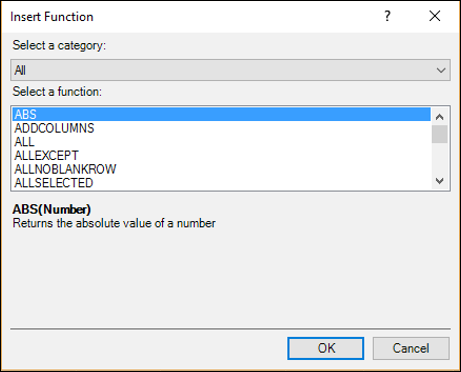## Using Insert Function in a DAX Formula

Suppose you want to create the following calculated field −

```Medal Count: = COUNTA (]Medal])
```

You can use Insert Function dialog box using the following steps −

• Click the calculation area of the Results table.
• Type the following in the formula bar −
```Medal Count: =
```
• Click the Insert Function button (fx).

Insert Function dialog box appears.

• Select Statistical in the Select a category box as shown in the following screenshot.

• Select COUNTA in the Select a function box as shown in the following screenshot.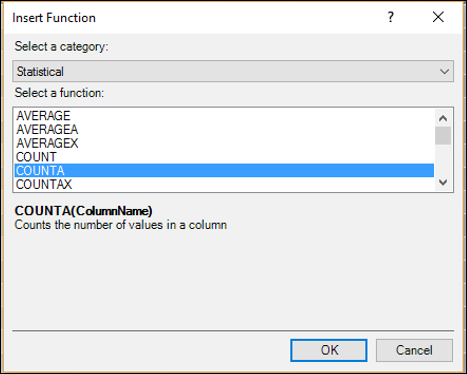As you can observe, the selected DAX function syntax and the function description are displayed. This enables you to make sure that it is the function that you want to insert.

• Click OK. Medal Count:=COUNTA( appears in the formula bar and a tooltip displaying the function syntax also appears.

• Type [. This means you are about to type a column name. The names of all the columns and the calculated fields in the current table will be displayed in the dropdown list. You can use IntelliSense to complete the formula.

• Type M. The displayed names in the dropdown list will be limited to those starting with ‘M’.

• Click Medal.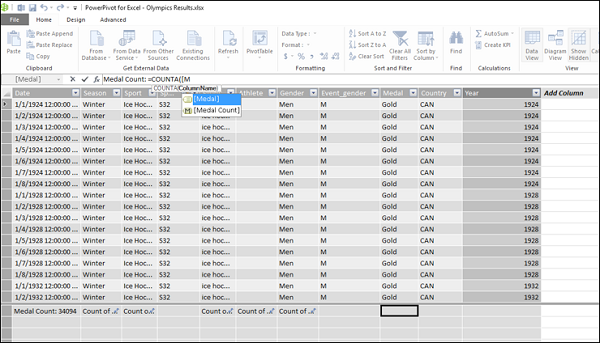• Double-click Medal. Medal Count: = COUNTA([Medal] will be displayed in the formula bar. Close the parenthesis.

• Press Enter. You are done. You can use the same procedure to create a calculated column also. You can also follow the same steps to insert a function in the Calculated Field dialog box in the Excel window using the Insert Function feature.

• Click the Insert Function (fx) button to the right of Formula.

Insert Function dialog box appears. The rest of the steps are the same as above.

## Using Multiple Functions in a DAX Formula

DAX formulas can contain up to 64 nested functions. But, it is unlikely that a DAX formula contains so many nested functions.

If a DAX formula has many nested functions, it has the following disadvantages −

• The formula would be very difficult to create.
• If the formula has errors, it would be very difficult to debug.
• The formula evaluation would not be very fast.

In such cases, you can split the formula into smaller manageable formulas and build the large formula incrementally.

## Creating a DAX Formula Using Standard Aggregations

When you perform data analysis, you will perform calculations on aggregated data. There are several DAX aggregation functions, such as SUM, COUNT, MIN, MAX, DISTINCTCOUNT, etc. that you can use in DAX formulas.

You can automatically create formulas using standard aggregations by using the AutoSum feature in the Power Pivot window.

• Click the Results tab in the Power Pivot window. Results table will be displayed.
• Click the Medal column. The entire column – Medal will be selected.
• Click the Home tab on the Ribbon.
• Click the down arrow next to AutoSum in the Calculations group.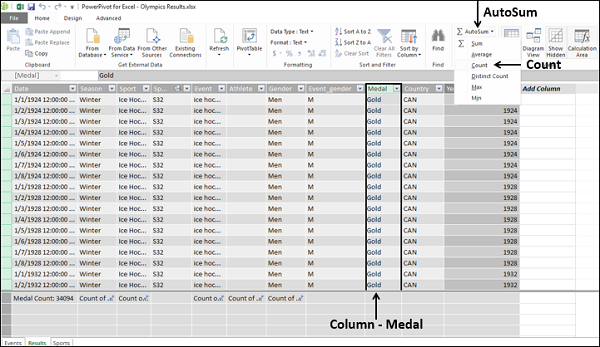• Click COUNT in the dropdown list.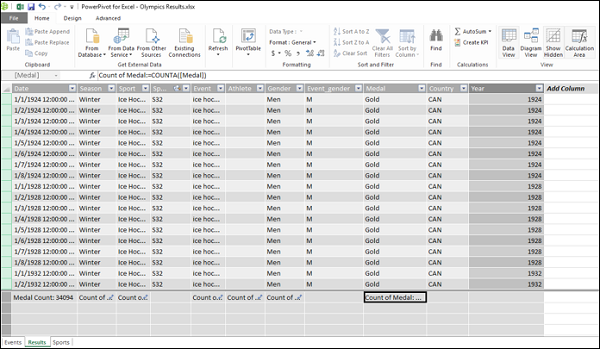As you can observe, the calculated field Count of Medal appears in the calculation area below the column – Medal. The DAX formula also appears in the formula bar −

```Count of Medal: = COUNTA([Medal])
```

The AutoSum feature has done the work for you – created the calculated field for data aggregation. Further, AutoSum has taken the appropriate variant of the DAX function COUNT, i.e. COUNTA (DAX has COUNT, COUNTA, COUNTAX functions).

A word of caution – To use AutoSum feature, you need to click the down arrow next to AutoSum on the Ribbon. If you click on the AutoSum itself instead, you will get −

```Sum of Medal: = SUM([Medal])
```

And an error is flagged as Medal is not a numeric data column and the text in the column cannot be converted to numbers.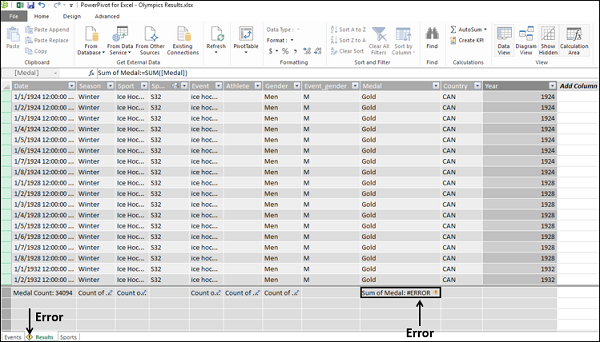You can refer to the chapter - DAX Error Reference for details on DAX errors.

## DAX Formulas and the Relational Model

As you are aware, in the Data Model of Power Pivot, you can work with multiple tables of data and connect the tables by defining relationships. This will enable you to create interesting DAX formulas that use the correlations of the columns among the related tables for calculations.

When you create a relationship between two tables, you are expected to make sure that the two columns used as keys have values that match, at least for most of the rows, if not completely. In the Power Pivot Data Model, it is possible to have non-matching values in a key column and still create a relationship, because Power Pivot does not enforce referential integrity (look at the next section for details). However, the presence of blank or non-matching values in a key column might affect the results of the DAX formulas and the appearance of PivotTables.

## Referential Integrity

Establishing referential integrity involves building a set of rules to preserve the defined relationships between tables when you enter or delete data. If you do not exclusively ensure this, as Power Pivot does not enforce it, you might not get correct results with the DAX formulas created before data changes are made.

If you enforce referential integrity, you can prevent the following pitfalls −

• Adding rows to a related table when there is no associated row in the primary table (i.e. with matching values in the key columns).

• Changing data in a primary table that would result in orphan rows in a related table (i.e. rows with a data value in the key column that does not have a matching value in the primary table key column).

• Deleting rows from a primary table when there are matching data values in the rows of the related table.

# Updating the Results of DAX Formulas

DAX formulas are used in calculations involving large data, including data from external data sources. The data can be subjected to changes from time to time as the DAX calculations are meant for live data as well.

The results of DAX formulas need to get updated on two occasions −

• Data Refresh − When the data is refreshed.

• Recalculation − When there are changes in the DAX formula.

## Understanding Data Refresh vs. Recalculation

Data refresh and recalculation are two separate but related operations.

• Data refresh is the process of updating the data in the Data Model in your workbook obtaining up-to-date data from external data sources.

• Recalculation is the process of updating all the columns, tables, and PivotTables in your workbook that contain DAX formulas, to reflect the changes in the underlying data that result from the changes to DAX formulas themselves.

You should not save or publish the workbook until the DAX formulas in it have been recalculated.

## Different Ways to Update Data in Data Model

Power Pivot does not automatically detect changes in external data sources.

• You can refresh data manually from the Power Pivot window at intervals that you can specify.

• You can schedule an automatic data refresh from external sources, if you have published the workbook to a SharePoint site.

For details on these, refer to the chapter – Updating Data in Data Model.

## Recalculation of DAX Formulas

Recalculation of a DAX formula is an important task, because during recalculation, column dependencies are checked and you will be notified if a column has changed, if the data is invalid, or if an error has appeared in a DAX formula that used to work.

Recalculation can affect performance in the following ways −

• For a calculated column, the result of DAX formula should always be recalculated for the entire column, whenever you change the DAX formula.

• For a calculated field, the result of DAX formula is not calculated until the calculated field is placed in the context of a PivotTable or a PivotChart. The DAX formula will be recalculated when you change any row or column heading that affects the filters on the data or when you manually refresh the PivotTable.

In DAX, recalculating formulas can be done automatically or manually.

# Excel DAX - Updating Data in the Data Model

DAX is used for calculations on the data in the Data Model in Excel Power Pivot. DAX enables data modeling and reporting activities to be handled in an effective way. However, this requires updating the data in the Data Model from time to time so as to reflect the current data.

You can import data from an external data source into the Data Model of your workbook by establishing a data connection. You can update the data from the source whenever you choose. This option is handy if you are getting data from relational databases that contain live sales information or data feeds that are updated several times a day.

## Different Ways of Updating Data in the Data Model

You can update the data in the Data Model in the following ways −

• Refreshing data in the Data Model from time to time.
• Making changes to data sources, such as connection properties.
• Updating the data in the Data Model after the source data has changed.
• Filtering the data to selectively load rows from a table in the data source.

## Refreshing Data in the Data Model

In addition to getting updated data from an existing source, you will need to refresh data in your workbook whenever you make changes to the schema of the source data. These changes can include adding columns or tables, or changing the rows that are imported.

Note that addition of data, changing data, or editing filters always triggers recalculation of DAX formulas that depend on that data source. Refer to the chapter – Recalculating DAX Formulas for details.

You have two types of data refresh in Data Model −

### Manual Refresh

If you choose manual refresh option, you can refresh the data in the Data Model manually at any time. You can refresh all data, which is the default, or you can manually choose the tables and columns to refresh for individual data sources.

### Automatic or Scheduled Refresh

If you have published your workbook to a PowerPivot Gallery or SharePoint site that supports PowerPivot, you or the SharePoint administrator can create a schedule for automatically updating the data in the workbook. In such a case, you can schedule unattended data refresh on the server.

## Manually Refreshing an Existing Data Source

You can manually refresh your data any time, if you need to update the data from an existing data source or get the recent data for designing new DAX formulas. You can refresh a single table, all tables that share the same data connection or all tables in the Data Model.

If you have imported data from a relational data source, such as SQL Server and Oracle, you can update all the related tables in one operation. The operation of loading new or updated data into the Data Model often triggers recalculation of DAX formulas, both of which might require some time to complete. Hence, you should be aware of the potential impact before you change data sources or refresh the data that is obtained from the data source.

To refresh data for a single table or all tables in a Data Model, do the following −

• Click the Home tab on the Ribbon in the Power Pivot window.
• Click Refresh.
• Click Refresh in the dropdown list for refreshing the selected table.
• Click Refresh All in the dropdown list for refreshing all the tables.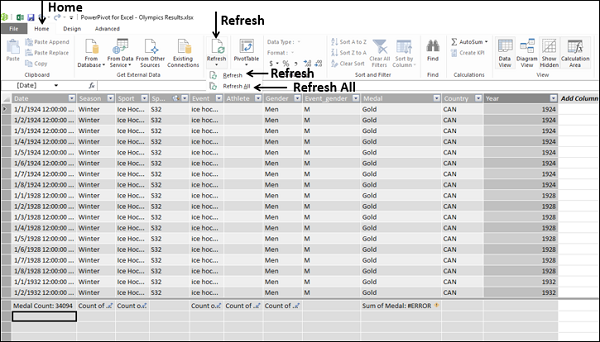To refresh data for all tables that use the same connection in a Data Model, do the following −

• Click the Home tab on the Ribbon in Power Pivot window.
• Click the Existing Connections in the Get External Data group.

Existing Connections dialog box appears.

• Select a connection.
• Click the Refresh button.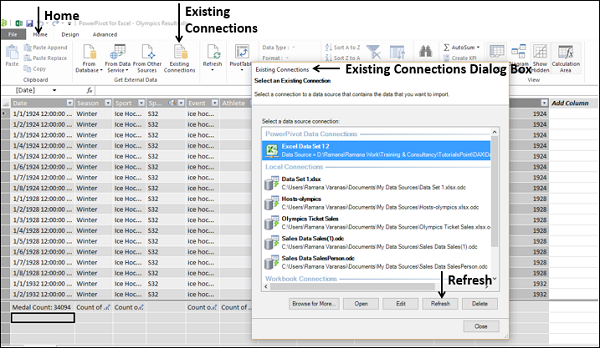Data Refresh dialog box appears and data refresh progress information is displayed as the PowerPivot engine reloads data from the selected table or from all tables from the data source.

There are three possible outcomes −

• Success − Reports on the number of rows imported into each table.

• Error − An error can occur if the database is offline, you no longer have permissions. A table or column is deleted or renamed in the source.

• Cancelled − This means Excel did not issue the refresh request, probably because refresh is disabled on the connection.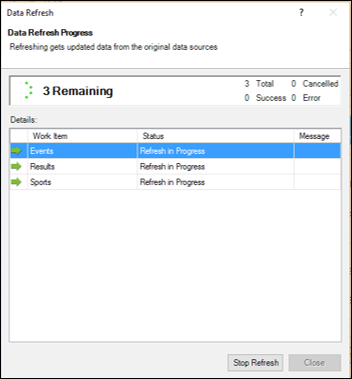Click the Close button.

## Changing a Data Source

To change the data in your Data Model, you can edit the connection information or update the definition of the tables and columns used in your Data Model in the Power Pivot window.

You can make the following changes to the existing data sources −

### Connections

• Edit the database name or the server name.
• Change the name of the source text file, spreadsheet, or data feed.
• Change the location of the data source.
• For relational data sources, change the default catalog or initial catalog.
• Change the authentication method or the credentials used to access the data.
• Edit advanced properties on the data source.

### Tables

• Add or remove a filter on the data.
• Change the filter criteria.
• Change the table names.
• Edit mappings between tables in the data source and tables in the Data Model.
• Select different columns from the data source.

### Columns

• Change the column names.
• Delete columns from the Data Model (does not affect the data source).

You can edit the properties of an existing data source in the following ways −

• You can change the connection information, including the file, feed, or database used as a source, its properties or other provider specific connection options.

• You can change the table and column mappings and remove references to columns that are no longer used.

• You can change the tables, views, or columns that you get from the external data source.

## Modifying a Connection to an Existing Data Source

You can modify the connection that you have created to an external data source by changing the external data source used by the current connection. However, the procedure to be followed depends on the data source type.

• Click the Home tab on the Ribbon in the PowerPivot window.
• Click the Existing Connections in the Get External Data group.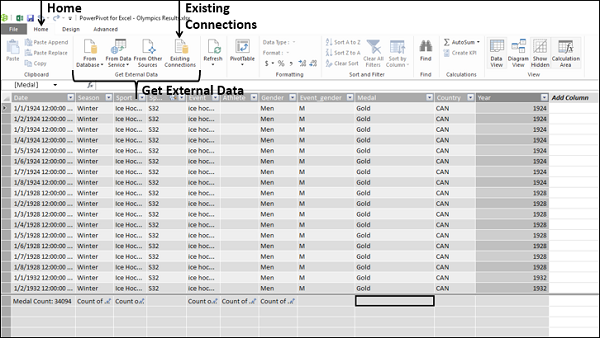Existing Connections dialog box appears. Select the connection that you want to modify.

Depending on the type of the data source you are changing, the provider might be different. Also the properties that are available may require change. Consider a simple example of a connection to an Excel workbook that contains the data.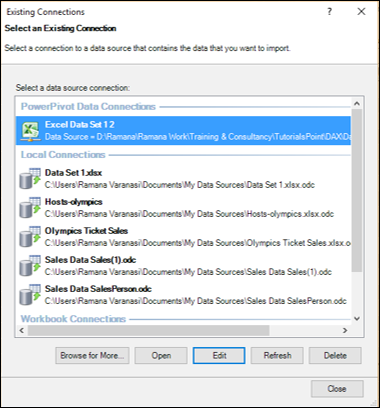• Click the Edit button. Edit Connection dialog box appears.

• Click the Browse button to locate another database of the same type (Excel workbook in this example), but with a different name or location.

• Click the Open button.

The new file will get selected. A message appears stating that you have modified connection information and you need to save and refresh the tables to verify the connection.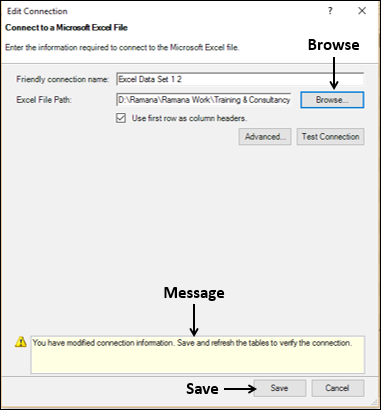• Click the Save button. You will be back in the Existing Connections dialog box.

• Click the Refresh button. Data Refresh dialog box appears displaying the data refresh progress. The status of data refresh will be displayed. Refer to the section - Manually Refreshing an Existing Data Source for details.

• Click Close, once the data refresh is a success.

• Click Close in the Existing Connections dialog box.

## Editing Table and Column Mappings (Bindings)

To edit the column mappings when a data source changes, do the following −

• Click the tab that contains the table you want to modify in the Power Pivot window.

• Click the Design tab on the Ribbon.

• Click the Table Properties.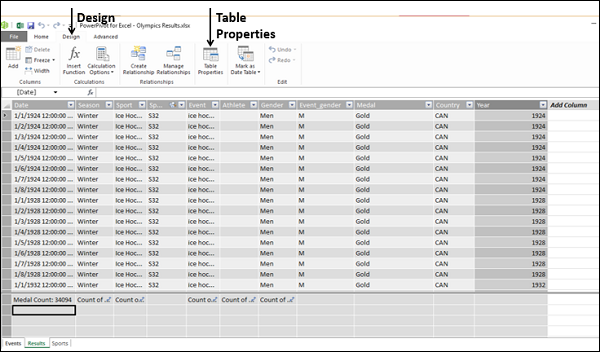Edit Table Properties dialog box appears.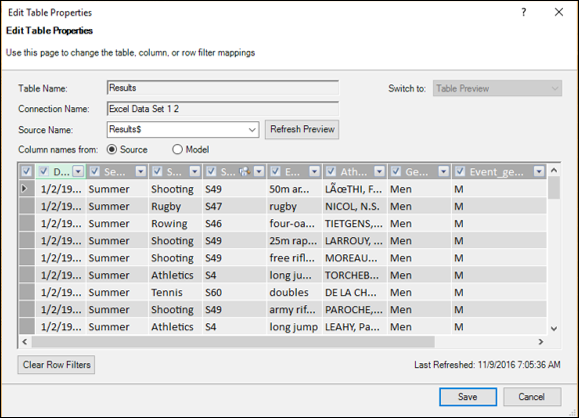You can observe the following −

• The name of the selected table in the Data Model is displayed in the Table Name box.

• The name of the corresponding table in the external data source is displayed in the Source Name box.

• There are two options for column names from – Source and Modal.

• If the columns are named differently in the data source and in the Data Model, you can toggle between the two sets of column names by selecting these options.

• Preview of the selected table appears in the dialog box.

You can edit the following −

• To change the table that is used as a data source, select a different table than the selected one in the Source Name dropdown list.

• Change the column mappings if needed −

• To add a column that is present in the source but not in the Data Model, select the checkbox beside the column name. Repeat for all the columns that are to be added. The actual data will be loaded into the Data Model, the next time you refresh.

• If some columns in the Data Model are no longer available in the current data source, a message appears in the notification area that lists the invalid columns. You do not need to do anything.

• Click the Save button.

When you save the current set of table properties, you will get a message – Please wait. Then the number of rows retrieved will be displayed.

In the table in the Data Model, any invalid columns are automatically removed and new columns are added.

## Changing a Column Name and Data Type

You can change the name of a column in a table in the Data Model as follows −

• Double-click on the header of the column. The name of the column in the header will get highlighted.

• Type the new column name, overwriting the old name. Alternatively, you can change the name of a column in a table in the Data Model as follows:

• Select the column by clicking on its header.

• Right-click the column.

• Click Rename Column in the dropdown list.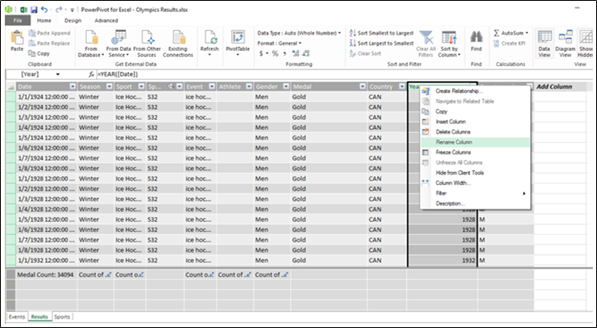The name of the column in the header will get highlighted. Type the new column name, overwriting the old name.

As you have learnt, all the values in a column in a table in the Data Model must be of the same data type.

To change the data type of a column, do the following −

• Select the column that you want to change by clicking its header.

• Click the Home tab on the Ribbon.

• Click the controls in the Formatting group to modify the column's data type and format.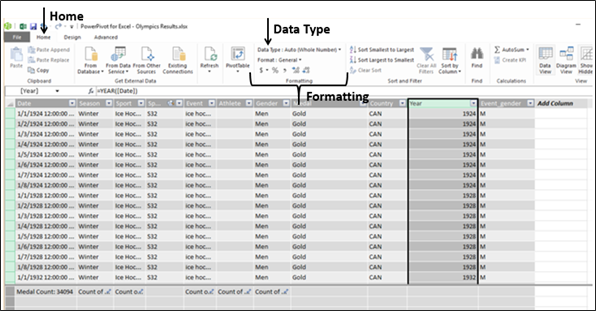## Adding / Changing a Filter to a Data Source

You can add a filter to a data source when you import data to restrict the number of rows in the table in the Data Model. Later, you can add more rows or decrease the number of rows in the table in the Data Model by changing the filter that you defined earlier.

### Adding a Filter to a Data Source During Import

To add a new filter to a data source during data import, do the following −

• Click the Home tab on the Ribbon in Power Pivot window.
• Click one of the data sources in the Get External Data group.

Table Import Wizard dialog box appears.

• Proceed to the step – Select Tables and Views.
• Select a table and then click Preview & Filter.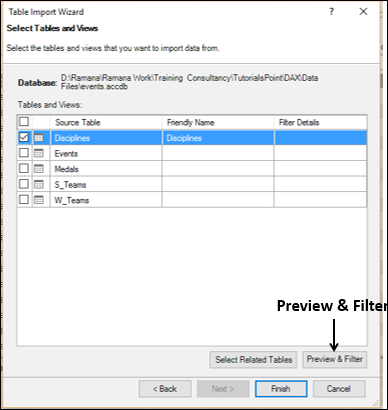Preview Selected Table dialog box appears.

• Click the column on which you want to apply filter.
• Click the down arrow to the right of the column heading.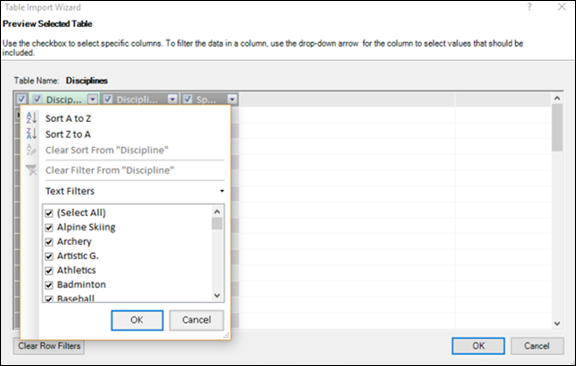To add a filter, do one of the following −

• In the list of column values, select or clear one or more values to filter by and then click OK.

However, if the number of values is extremely large, individual items might not be shown in the list. Instead, you will see the message - "Too many items to show."

• Click Number Filters or Text Filters (depending on the data type of the column).

• Then, click one of the comparison operator commands (such as Equals), or click Custom Filter. In the Custom Filter dialog box, create the filter and then click OK.

Note − If you make a mistake at any stage, click the Clear Row Filters button and start over.

• Click OK. You will be back to Select Tables and Views page of Table Import Wizard.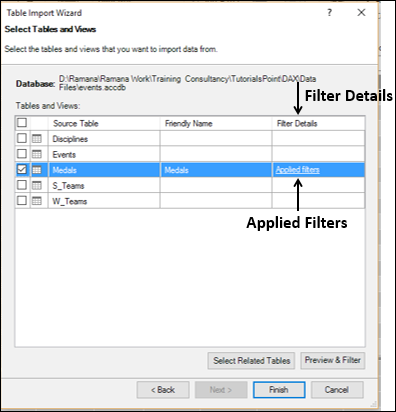As you can observe, in the column – Filter Details, a link Applied Filters appears for the column on which you defined the filter.

You can click the link to view the filter expression that was built by the wizard. But, the syntax for each filter expression depends on the provider and you cannot edit it.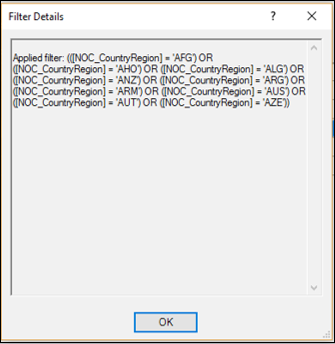• Click Finish to import the data with filters applied.
• Close the Table Import Wizard.

### Changing a Filter to an Existing Data Source

After you have imported the data, you might have to update it from time to time, by either adding more rows or by restricting the existing rows in the table. In such a case, you can change the existing filters on the table or add new filters.

• Click the Home tab on the Ribbon in Power Pivot window.

• Click the Existing Connections in the Get External Data group. Existing Connections dialog box appears.

• Click the connection that contains the table on which you have to change the filter.

• Click the Open button.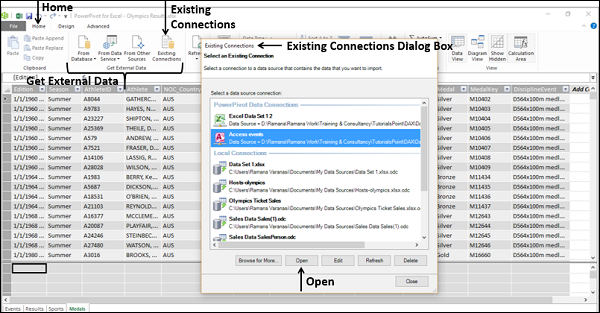You will get into Table Import Wizard dialog box. Repeat the steps in the previous section to filter the columns.

# Excel DAX - Recalculating DAX Formulas

Recalculation of a DAX formula is required to reflect changes in the data and changes in the formula itself. However, recalculating a DAX formula involves performance cost.

Even then, to obtain accurate results, recalculation is essential. During recalculation, column dependencies are checked and you will be notified if a column has changed, if the data is invalid or if an error has appeared in a DAX formula that used to work.

## Types of Recalculation

You have two options for recalculating DAX formulas −

• Automatic Recalculation Mode (default)
• Manual Recalculation Mode

By default, Power Pivot automatically recalculates as required while optimizing the time required for processing. However, you can choose to update calculations manually, if you are working with complex formulas or very large data sets and want to control the timing of updates.

Both automatic and manual modes of recalculating DAX formulas have advantages. However, the recommended way is to use automatic recalculation mode. This way you can keep the Power Pivot data in sync and prevent problems caused by deletion of data, changes in names or data types or missing dependencies.

## Recalculating DAX Formulas Automatically

If you choose the default mode of recalculating DAX formulas, i.e. recalculating automatically, any changes to data that would cause the result of any DAX formula to change will trigger recalculation of the entire column that contains the DAX formula.

The following changes always require recalculation of DAX formulas −

• Values from an external data source have been refreshed.

• The DAX formula itself is changed.

• Names of tables or columns that are referenced in the DAX formula have been changed.

• Relationships between tables have been added, modified or deleted.

• New calculated fields or calculated columns have been added.

• Changes have been made to other DAX formulas within the workbook, so columns or calculations that depend on those DAX formulas need to be recalculated.

• Rows have been inserted or deleted in the table.

• You applied a filter that requires execution of a query to update the data set. The filter could have been applied either in a DAX formula or as part of a PivotTable or PivotChart.

## When to Use Manual Recalculation Mode?

You can use manual recalculation mode until you are ready with all your required DAX formulas in your workbook. This way, you can avoid incurring the cost of computing formula results on the workbook that is still in the draft state.

You can use manual recalculation of DAX formulas in the following conditions −

• You are designing a DAX formula by using a template and want to change the names of the columns and tables used in the DAX formula before you validate it.

• You know that some data in the workbook has changed but you are working with a different column that has not changed so you want to postpone a recalculation.

• You are working in a workbook that has many dependencies and want to defer recalculation till you are sure that all the necessary changes have been made.

But, you should be aware that as long as the workbook is configured to manual recalculation mode, any validation or checking of formulas is not performed. This will result in the following −

• Any new formulas that you add to the workbook will be flagged as containing an error.

## Configuring the Workbook for Manual Recalculation

As you have learnt, automatic recalculation is the default mode in the Data Model of any workbook. To configure a workbook for manual recalculation, do the following −

• Click the Design tab on the Ribbon in the Power Pivot window.
• Click the Calculation Options in the Calculations group.
• Click the Manual Calculation Mode in the dropdown list.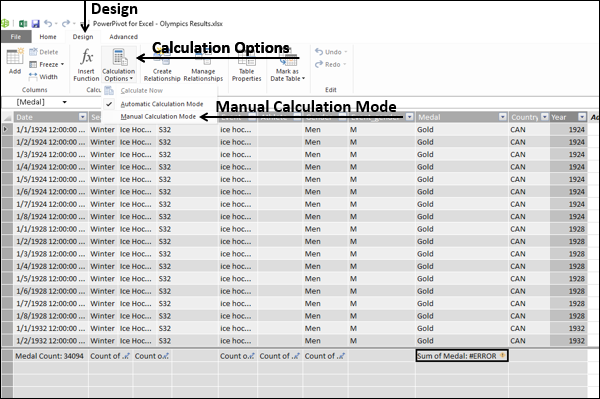## Recalculating DAX Formulas Manually

To recalculate the DAX formulas manually, do the following −

• Click the Design tab on the Ribbon in the Power Pivot window.
• Click the Calculation Options field in the Calculations group.
• Click the Calculate Now field in the dropdown list.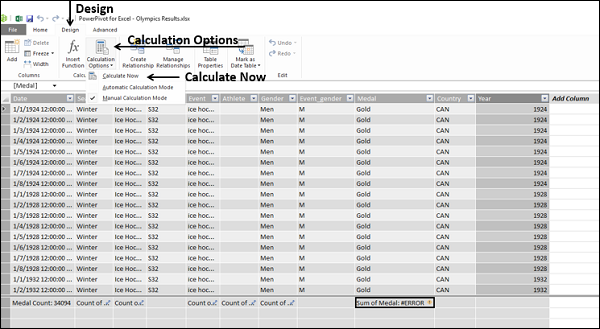# Troubleshooting DAX Formula Recalculation

Whenever changes occur in the Data Model of your workbook, Power Pivot performs an analysis of the existing data to determine whether recalculation is required and performs the update in the most efficient way possible.

Power Pivot handles the following, during recalculation of DAX formulas −

• Dependencies
• Sequence of recalculation for dependent columns
• Transactions
• Recalculation of volatile functions

## Dependencies

When a column depends on another column, and the contents of that other column change in any way, all related columns might need to be recalculated.

Power Pivot always performs a complete recalculation for a table, because a complete recalculation is more efficient than checking for changed values. The changes that trigger recalculation might include deleting a column, changing the numeric data type of a column or adding a new column. These changes are considered as major changes. However, seemingly trivial changes, such as changing the name of a column might also trigger recalculation. This is because the names of the columns are used as identifiers in the DAX formulas.

In some cases, Power Pivot may determine that columns can be excluded from recalculation.

## Sequence of Recalculation for Dependent Columns

Dependencies are calculated prior to any recalculation. If there are multiple columns that depend on each other, Power Pivot follows the sequence of dependencies. This ensures that the columns are processed in the right order at the maximum speed.

## Transactions

Operations that recalculate or refresh data take place as a transaction. This means that if any part of the refresh operation fails, the remaining operations are rolled back. This is to ensure that data is not left in a partially processed state. However, you cannot manage the transactions as you do in a relational database or create checkpoints.

## Recalculation of Volatile Functions

DAX functions such as NOW, RAND, or TODAY do not have fixed values and are referred to as volatile functions. If such DAX functions are used in a calculated column, the execution of a query or filtering will usually not cause them to be re-evaluated to avoid performance problems.

The results for these DAX functions are only recalculated when the entire column is recalculated. These situations include refresh from an external data source or manual editing of data that causes re-evaluation of DAX formulas that contain these functions.

However, such functions will always be recalculated if the functions are used in the definition of a Calculated Field.

# Excel DAX - Formula Errors

You can get errors when you write DAX formulas with wrong syntax. Calculated fields and calculated columns can contain DAX functions that require a specific type of arguments. Arguments of DAX functions can be tables, columns, or other DAX functions (nested DAX functions). As DAX functions can return tables and columns, care should be taken to check that the right type of arguments are passed to the DAX functions.

DAX formula errors can be either syntax errors or semantic errors. The errors can occur either at design time or at run time.

In this chapter, you will learn about some common DAX errors, their causes, and how to fix those errors.

## DAX Error: Calculation Aborted

The following error can occur when attempting to create (design-time) or use (run-time) a calculated field with a DAX time-intelligence function. In each case, a noncontiguous date range is being passed to the time intelligence function.

“DAX Error: CALCULATION ABORTED: MdxScript (instance) (00, 0) Function ‘DATEADD’ only works with contiguous date selections.”

### Cause at Run-time

This error can be displayed when a calculated field with a DAX time intelligence function is placed in the VALUES area of a PivotTable and date fields such as the month or the quarter are selected as slicers or filters before selecting a year. For example, if you have data of three years – 2014, 2015, and 2016 and you try to use only the month March without selecting the Year field, then the values are not contiguous data values and you will get an error.

### How to Fix the Error at Run-time?

In the above example,

• First add Year as a slicer or a filter and select a year.

• Then, add Month or Quarter as a slicer or a filter.

• Then, select one or more months or quarters to slice or filter on for the year selected.

### Cause at Design-time

DAX time intelligence functions require a date column specified for the date argument. The date column must have a contiguous range of dates. This error can be returned, if there is a date value in one or more rows in the date column that is not contiguous with the data values in the previous and successive rows.

If you imported your table containing dates from a data source, remember that many organizations run special processes that scan tables in databases for invalid values and replace those with a particular value. That is, if an invalid date is found, it is assigned a particular date value that may not be contiguous with other data values in the column.

### How to Fix This Error at Design-time?

Do the following to fix the error at design time −

• If your date table is imported from a data source, use Refresh in Power Pivot window to reimport any changes found at the source.

• Check the values in your date column to make sure they are in a contiguous order. If any value is found not to be in place, it will have to be corrected at the source and the date table will have to be refreshed.

• Create a separate date table and date column in your Data Model. Specify the new date column as the date argument in the formula causing the error. Date tables are easy to create and add to a Data Model.

## DAX Semantic Error - An Example

The following DAX error is a semantic error −

“A function ‘CALCULATE’ has been used in a true-false expression that is used as a table filter expression. This is not allowed.”

### Cause

This error can appear when one or more filter expressions cannot be used in context of the calculated field or calculated column expression.

In most of the cases, this error is caused by a filter expression specified as an argument to the DAX CALCULATE function. The CALCULATE function requires filters defined as a Boolean expression or a table expression.

### How to Fix Such Errors?

You can fix such errors by using the DAX FILTER function to define filters as a table expression, which can then be used as an argument to the DAX CALCULATE function.

# Excel DAX - Time Intelligence

DAX has an important and powerful feature, referred to as Time Intelligence. Time intelligence enables you to write DAX formulas that refer to the time periods for use in the PivotTables.

DAX has 35 time-intelligence functions specifically for aggregating and comparing data over time. However, these DAX functions have some constraints on the data that you need to understand and work with caution to avoid errors.

## Why Time Intelligence Makes DAX Powerful?

The time intelligence functions work with data that is constantly changing, depending on the context you select in PivotTables and Power View visualizations. As you are aware, most of the data analysis involves summarization of data over time periods, comparing data values across the time periods, understanding the trends and making decisions based on future projections.

For example, you might want to sum sales amounts for the past month product-wise and compare the totals with those of other months in the fiscal year. This means, you have to use the dates as a way to group and aggregate sales transactions for a particular period in time.

This is where you can observe the power of DAX. You can use DAX time intelligence functions to define calculated fields that help you in analyzing the data over time, without having to change the date selections in the pivot tables. This makes your job easier. Moreover, you can build PivotTables that would not be possible any other way.

## Requirements for DAX Time Intelligence Functions

DAX time intelligence functions have certain requirements. If these requirements are not met, you might get errors or they may not work properly. Hence, you can refer to these requirements as rules or constraints as well. Following are certain DAX time intelligence functions requirements/rules/constraints −

• You need to have a date table in your Data Model.

• The date table must include a column considered to be the Date column by DAX. You can name the column the way you want, but it should comply with the following conditions: o The date column should contain a contiguous set of dates that covers every day in the time period you are analyzing the data.

• Every date must exist once and only once in the date column.

• You cannot skip any dates (For e.g. you cannot skip weekend dates).

• DAX time intelligence functions work only on a standard calendar and assume the start of the year as January 1 and the end of the year as December 31, with the months in the year and days in each month as of a calendar year.

However, you can customize a standard calendar for different financial years. It is a good practice to verify the above requirements before any time intelligence function is used.

For more details on date tables and their usage in DAX formulas, refer to the tutorial = Data Modeling with DAX in this tutorials library.

## DAX Time Intelligence Functions – Categories

DAX Time Intelligence functions can be categorized as follows −

• DAX functions that return a single date.
• DAX functions that return a table of dates.
• DAX functions that evaluate expressions over a time period.

### DAX Functions That Return a Single Date

DAX functions in this category return a single date.

There are 10 DAX functions in this category −

Sr.No. DAX Function & Return Value
1

FIRSTDATE (Date_Column)

Returns the first date in the Date_Column in the current context.

2

LASTDATE (Date_Column)

Returns the last date in the Date_Column in the current context.

3

FIRSTNONBLANK (Date_Column, Expression)

Returns the first date where an expression has a non-blank value.

4

LASTNONBLANK (Date_Column, Expression)

Returns the last date where an expression has a non-blank value.

5

STARTOFMONTH (Date_Column)

Returns the first date of a month in the current context.

6

ENDOFMONTH (Date_Column)

Returns the last date of a month in the current context.

7

STARTOFQUARTER (Date_Column)

Returns the first date of a quarter in the current context.

8

ENDOFQUARTER (Date_Column)

Returns the last date of a quarter in the current context.

9

STARTOFYEAR (Date_Column, [YE_Date])

Returns the first date of a year in the current context.

10

ENDOFYEAR (Date_Column, [YE_Date])

Returns the last date of a year in the current context.

### DAX Functions That Return a Table of Dates

DAX Functions in this category return a table of dates. These functions will be mostly used as a SetFilter argument to the DAX function - CALCULATE.

There are 16 DAX functions in this category. Eight (8) of these DAX functions are the “previous” and “next” functions.

• The “previous” and “next” functions start with a date column in the current context and calculate the previous or next day, month, quarter or year.

• The “previous” functions work backward from the first date in the current context and the “next” functions move forward from the last date in the current context.

• The “previous” and “next” functions return the resulting dates in the form of a single column table.

Sr.No. DAX Function & Return Value
1

PREVIOUSDAY (Date_Column)

Returns a table that contains a column of all dates representing the day that is previous to the first date in the Date_Column in the current context.

2

NEXTDAY (Date_Column)

Returns a table that contains a column of all dates from the next day, based on the first date specified in the Date_Column in the current context.

3

PREVIOUSMONTH (Date_Column)

Returns a table that contains a column of all dates from the previous month, based on the first date in the Date_Column in the current context.

4

NEXTMONTH (Date_Column)

Returns a table that contains a column of all dates from the next month, based on the first date in the Date_Column in the current context.

5

PREVIOUSQUARTER (Date_Column)

Returns a table that contains a column of all dates from the previous quarter, based on the first date in the Date_Column in the current context.

6

NEXTQUARTER (Date_Column)

Returns a table that contains a column of all dates in the next quarter, based on the first date specified in the Date_Column in the current context.

7

PREVIOUSYEAR (Date_Column, [YE_Date])

Returns a table that contains a column of all dates from the previous year, given the last date in the Date_Column in the current context.

8

NEXTYEAR (Date_Column, [YE_Date])

Returns a table that contains a column of all dates in the next year, based on the first date in the Date_Column in the current context.

Four (4) DAX functions calculate a set of dates in a period. These functions perform the calculations using the last date in the current context.

Sr.No. DAX Function & Return Value
1

DATESMTD (Date_Column)

Returns a table that contains a column of the dates for the month to date, in the current context.

2

DATESQTD (Date_Column)

Returns a table that contains a column of the dates for the quarter to date, in the current context.

3

DATESYTD (Date_Column, [YE_Date])

Returns a table that contains a column of the dates for the year to date, in the current context.

4

SAMEPERIODLASTYEAR (Date_Column)

Returns a table that contains a column of dates shifted one year back in time from the dates in the specified Date_Column, in the current context.

Note− SAMEPERIODLASTYEAR requires that the current context contains a contiguous set of dates.

If the current context is not a contiguous set of dates, then SAMEPERIODLASTYEAR will return an error.

• Four (4) DAX functions are used to shift from the set of dates that are in the current context to a new set of dates.

These DAX functions are more powerful than the previous ones.

• DAX functions – DATEADD, DATESINPERIOD and PARALLELPERIOD shift some number of time intervals from the current context. The interval can be day, month, quarter or year, represented by the key words – DAY, MONTH, QUARTER and YEAR respectively.

For example:

• Shift backward by 2 days.

• Move forward by 5 months.

• Move forward by one month from today.

• Go back to same quarter in the last year.

If the function argument - number of intervals (integer value) is positive, shift is forward and if it is negative, shift is backward.

• DAX function – DATESBETWEEN calculates the set of dates between the specified start date and the end date.

Sr.No. DAX Function & Return Value
1

Returns a table that contains a column of dates, shifted either forward or backward in time by the specified number of intervals from the dates in the current context.

2

DATESINPERIOD (Date_Column, Start_Date, Number_of_Intervals, Interval)

Returns a table that contains a column of dates that begins with the start_date and continues for the specified number_of_intervals.

3

PARALLELPERIOD (Date_Column, Number_of_Intervals, Interval)

Returns a table that contains a column of dates that represents a period parallel to the dates in the specified Date_Column in the current context, with the dates shifted a number of intervals either forward or backward in time.

4

DATESBETWEEN (Date_Column, Start_Date, End_Date)

Returns a table that contains a column of dates that begins with the start_date and continues until the end_date.

### DAX Functions that Evaluate Expressions Over a Time Period

DAX Functions in this category evaluate an expression over a specified time period.

There are nine (9) DAX functions in this category −

• Three (3) DAX functions in this category can be used to evaluate any given expression over a specified time period.

Sr.No. DAX Function & Return Value
1

TOTALMTD (Expression, Date_Column, [SetFilter])

Evaluates the value of the expression for the dates in the month to date, in the current context.

2

TOTALQTD (Expression, Date_Column, [SetFilter])

Evaluates the value of the expression for the dates in the quarter to date, in the current context.

3

TOTALYTD (Expression, Date_Column, [SetFilter], [YE_Date])

Evaluates the value of the expression for the dates in the year to date, in the current context

• Six (6) DAX functions in this category can be used to calculate the opening and the closing balances.

• The opening balance for any period is the same as the closing balance for the previous period.

• The closing balance includes all data through the end of the period, while the opening balance does not include any data from within the current period.

• These DAX functions always return the value of an expression evaluated for a specific point in time.

• The point in time we care about is always the last possible date value in a calendar period.

• The opening balance is based on the last date of the previous period, while the closing balance is based on the last date in the current period.

• The current period is always determined by the last date in the current date context.

Sr.No. DAX Function & Return Value
1

OPENINGBALANCEMONTH (Expression, Date_Column, [SetFilter])

Evaluates the expression at the first date of the month in the current context.

2

CLOSINGBALANCEMONTH (Expression, Date_Column, [SetFilter])

Evaluates the expression at the last date of the month in the current context.

3

OPENINGBALANCEQUARTER (Expression, Date_Column, [SetFilter])

Evaluates the expression at the first date of the quarter, in the current context.

4

CLOSINGBALANCEQUARTER (Expression, Date_Column, [SetFilter])

Evaluates the expression at the last date of the quarter in the current context.

5

OPENINGBALANCEYEAR (Expression, Date_Column, [SetFilter], [YE_Date])

Evaluates the expression at the first date of the year in the current context.

6

CLOSINGBALANCEYEAR (Expression, Date_Column, [SetFilter], [YE_Date])

Evaluates the expression at the last date of the year in the current context.

# Excel DAX - Filter Functions

DAX has powerful filter functions that are quite different from Excel functions. The lookup functions work by using tables and relationships, like a database. The filtering functions let you manipulate data context to create dynamic calculations.

Note − DAX filter functions that return a table do not add the table to the Data Model. The resulting table is used as an argument in another DAX function. That is, such DAX functions are used as nested functions with other DAX functions.

In the next section, you will learn what DAX filter functions you can use. For more details on these functions, refer to the tutorial – DAX Functions in this tutorials library.

## DAX Filter Functions

Following are the DAX Filter functions −

Sr.No. DAX Function & What the Function Does?
1

ADDMISSINGITEMS (<showAllColumn>, [<showAllColumn>] …, <table>, <groupingColumn>, [<groupingColumn>] …, [filterTable] …)

Adds combinations of items from multiple columns to a table if they do not already exist. The determination of which item combinations to add is based on referencing source columns which contain all the possible values for the columns.

To determine the combinations of items from different columns to evaluate −

• AutoExist is applied for columns within the same table.
• CrossJoin is applied across different tables.
2

ALL ( {<table> | <column>, [<column>], [<column>], … })

Returns all the rows in the given table or all the values in the specified columns in a table, ignoring any filters that might have been applied.

This function is useful for clearing filters and creating calculations on all the rows in a table.

3

ALLEXCEPT (<table>, <column>, [<column>], …)

Removes all context filters in the table except filters that have been applied to the columns specified as arguments.

As against ALL, you can use this function when you want to remove the filters on many, but not all, columns in a table.

4

ALLNOBLANKROW (<table>|<column>)

From the parent table of a relationship, returns −

• all rows but the blank rows, or
• all distinct values of a column but the blank rows

The function disregards any context filters that might exist.

5

ALLSELECTED ( [<tableName>|<columnName>] )

Removes context filters from columns and rows in the current query, while retaining all other context filters or explicit filters.

6

CALCULATE (<expression>, [<filter1>, <filter2> …)]

Evaluates an expression in a context that is modified by the specified filters.

Returns the value that is the result of the expression.

7

CALCULATETABLE (<expression>, <filter1>, <filter2>, …)

Evaluates a table expression in a context modified by the given filters.

Returns a table of values.

8

CROSSFILTER (<columnName1>, <columnName2>, <direction>)

Specifies the cross-filtering direction to be used in a calculation for a relationship that exists between two columns. Does not return any value.

9

DISTINCT (<column>)

Returns a one-column table that contains the distinct values from the specified column. In other words, duplicate values are removed and only unique values are returned.

The resulting column is used as an argument in another DAX function.

10

EARLIER (<column>, <number>)

Returns the current value of the specified column in an outer evaluation pass of the mentioned column specified by the number.

11

EARLIEST (<column>)

Returns the current value of the specified column in an outer evaluation pass of the specified column.

12

FILTER (<table>, <filter>)

Returns a table that contains only the filtered rows.

FILTER is used only as a function that is embedded in other functions that require a table as an argument.

13

FILTERS (<columnName>)

Returns the values that are directly applied as filters to columnName.

FILTERS is used only as a function that is embedded in other functions that require a table as an argument.

14

HASONEFILTER (<columnName>)

Returns TRUE when the number of directly filtered values on columnName is one. Otherwise, returns FALSE.

15

HASONEVALUE (<columnName>)

Returns TRUE when the context for columnName has been filtered down to one distinct value only. Otherwise, returns FALSE.

16

ISCROSSFILTERED (<columnName>)

Returns TRUE when columnName or another column in the same or related table is being filtered.

17

ISFILTERED (<columnName>)

Returns TRUE when columnName is being filtered directly. If there is no filter on the column or if the filtering happens because a different column in the same table or in a related table is being filtered, then the function returns FALSE.

18

KEEPFILTERS (<expression>)

Modifies how filters are applied while evaluating a CALCULATE or CALCULATETABLE function.

19

RELATED (<column>)

Returns a related value from another table.

20

RELATEDTABLE (<tableName>)

Evaluates a table expression in a context modified by the given filters.

21

SUBSTITUTEWITHINDEX (<table>, <indexColumnName>, <indexColumnTable>, <orderBy_expression>, [<order>])

Returns a table which represents a left semijoin of the two tables supplied as arguments.

The semijoin is performed by using common columns, determined by common column names and common data type.

The columns being joined on are replaced with a single column in the returned table which is of type integer and contains an index.

The index is a reference into the right join table given a specified sort order.

22

USERELATIONSHIP ( <columnName1>,<columnName2>)

Specifies the relationship to be used in a specific calculation as the one that exists between columnName1 and columnName2.

23

VALUES (<TableNameOrColumnName>)

Returns a one-column table that contains the distinct values from the specified table or column.

In other words, duplicate values are removed and only unique values are returned.

# Excel DAX - Scenarios

You have learnt DAX syntax, the usage of DAX operators and DAX functions in the previous chapters. As you are aware, DAX is a formula language used for data modeling and data analysis.

DAX can be used in various scenarios. Based on the DAX scenarios, DAX optimizes the performance and produces accurate and effective results. In this chapter, you will get to know some of the DAX scenarios.

## Performing Complex Calculations

DAX formulas can perform complex calculations that involve custom aggregations, filtering, and the use of conditional values. You can do the following with DAX

• Create custom calculations for a PivotTable.
• Apply a filter to a formula.
• Remove filters selectively to create a dynamic ratio.
• Use a value from an outer loop.

For details, refer to the chapter Scenarios - Performing Complex Calculations.

## Working with Text and Dates

DAX can be used in the scenarios involving working along with text, extracting and composing date and time values, or creating values based on a condition. You can do the following with DAX −

• Create a key column by concatenation.
• Compose a date based on date parts extracted from a text date.
• Define a custom date.
• Change data types using a formula.
• Convert real numbers to integers.
• Convert real numbers, integers, or dates to strings.
• Convert strings to real numbers or dates.

For details, refer to the chapter Scenarios - Working with Text and Dates.

## Conditional Values and Testing for Errors

DAX functions enable to test values in the data and return a different value based on a condition. DAX functions that test values are also useful for checking the range or type of values, to prevent unexpected data errors from breaking calculations. You can do the following with DAX −

• Create a value based on a condition.
• Test for errors within a formula.

For details, refer to the chapter Scenarios - Conditional Values and Testing for Errors.

## Using Time Intelligence

You have learnt about DAX time intelligence functions in the chapter – Understanding DAX time intelligence.

DAX time intelligence functions include functions to help you retrieve dates or date ranges from your data. You can then use those dates or date ranges to calculate values across similar periods. The time intelligence functions also include functions that work with standard date intervals, to allow you to compare values across months, years, or quarters. You could also create a DAX formula that compares values for the first and the last date of a specified period.

You can learn more about DAX intelligence functions and what they can do for the following −

• Calculate Cumulative Sales.
• Compare Values over Time.
• Calculate a Value over a Custom Date Range.

For details, refer to the chapter Scenarios - Using Time Intelligence.

## Ranking and Comparing Values

If you want to show only the top n number of items in a column or a PivotTable, you have the following options −

• Apply a filter to show only the top or bottom few items.
• Create a DAX formula that dynamically ranks values and apply a filter.

Each of these options have pros and cons.

For details, refer to the chapter Scenarios - Ranking and Comparing Values.

# Excel DAX - Performing Complex Calculations

DAX formulas can perform complex calculations that involve custom aggregations, filtering, and the use of conditional values. You can do the following with DAX −

• Create custom calculations for a PivotTable.
• Apply a filter to a DAX formula.
• Remove filters selectively to create a dynamic ratio.
• Use a value from an outer loop.

## Creating Custom Calculations for a PivotTable

DAX functions CALCULATE and CALCULATETABLE are powerful and flexible. They are useful for defining calculated fields. These DAX functions enable you to change the context in which the calculation will be performed. You can also customize the type of aggregation or mathematical operation to perform.

### CALCULATE Function

CALCULATE (<expression>, [<filter1>], [<filter2>]…)

CALCULATE function evaluates the given expression in a context that is modified by zero or more specified filters.

If your data has been filtered, the CALCULATE function changes the context in which the data is filtered and evaluates the expression in the new context that you specify by the filters. That means, any existing filters on the specified column are removed and the filter used in the filter argument is applied instead.

### Example

Suppose you want to display the percentage of medals sport-wise filtered by Country names. Your calculation should get the percentage value overriding the filter that you apply on Country in the PivotTable.

• Define a calculated field – Percentage of Medal Count as shown in the following screenshot.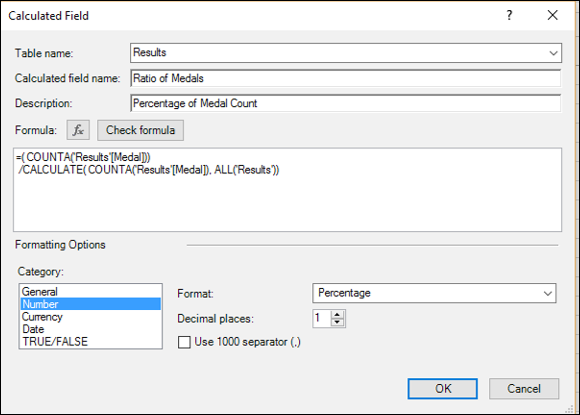With this DAX formula, all the rows in the Results table are taken into account in the CALCULATE function with the filter containing the ALL function. This way, you have the total count in the denominator.

Your PivotTable will be as shown in the following screenshot.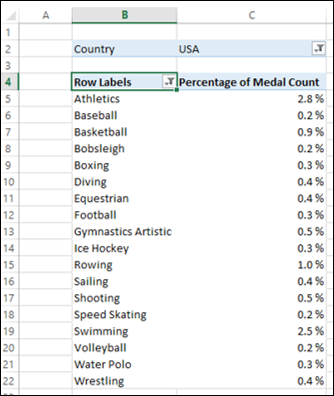In the above screenshot, Country is filtered to USA and Top 18 values are displayed in the PivotTable. Next, you can dynamically filter values in the PivotTable. However, the calculations will be correct by the custom DAX formula that you used.

The CALCULATETABLE function takes a table of values and performs the same action as that of CALCULATE function.

## Filtering Data in Formulas

You can create filters within DAX formulas, to select the values from the source data for use in calculations. You can do this by defining a filter expression and using it along with the table that is an input to the DAX formula.

The filter expression enables you to obtain a subset of the source data. The filter is applied dynamically each time that you update the results of the DAX formula, depending on the current context of your data and you can be assured of accurate and expected results.

The filter expression typically contains a DAX filter function that returns only selected rows of the table, which then can be used as an argument for another DAX function that you are using for data aggregation.

### Example

The following screenshot shows the definition of a calculated field that gives medal count only for summer sports.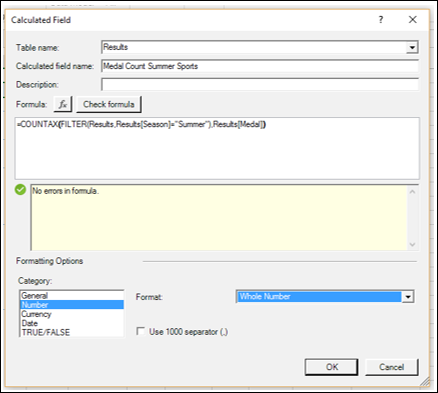With this calculated field, the PivotTable looks as shown in the following screenshot.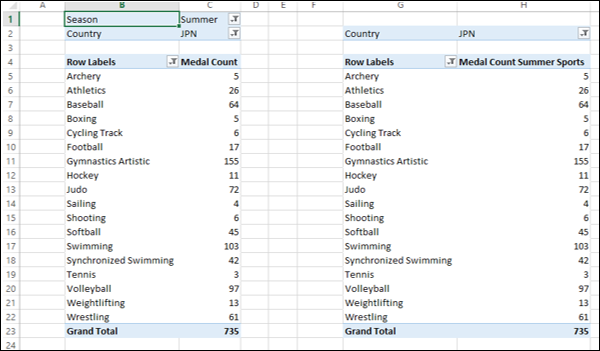As you can observe, the values in the PivotTable on the right side with the new calculated field match with those that are in the PivotTable on the left side with the filter on Season field applied explicitly.

Note − DAX filter and value functions return a table, but never return the table or rows directly to the Data Model and hence are always embedded in another DAX function.

For details on these DAX functions, refer to the chapter – DAX Filter Functions.

### Adding and Removing Filters Dynamically

DAX Formulas that you use in a PivotTable can be affected by the PivotTable context. However, you can selectively change the context by adding or removing filters. You can use the DAX functions ALL and ALLEXCEPT to dynamically select the rows irrespective of the PivotTable context.

Additionally, you can use the DAX functions DISTINCT and VALUES for returning distinct values.

### Using a Value from an Outer Loop

You can use a value from a previous loop in creating a set of related calculations with DAX EARLIER function. This DAX function supports up to two levels of nested loops.

# Excel DAX - Working with Text and Dates

DAX can be used in the scenarios involving working along with text, extracting and composing date and time values or creating values based on a condition. You can do the following with DAX −

• Create a key column in a table by concatenation.
• Compose a date based on date parts extracted from a text date.
• Define a custom date format.
• Change data types using a formula.
• Convert real numbers to integers.
• Convert real numbers, integers, or dates to strings.
• Convert strings to real numbers or dates.

## Creating a Key Column by Concatenation

Data Model in PowerPivot allows only a single key column. It does not support composite keys that you might find in the external data sources. Hence, if any composite keys exist in a table in the data source, you need to combine them into a single key column for the table in the Data Model.

You can use the DAX function CONCATENATE to combine two columns into a single column in a table in the Data Model. DAX function CONCATENATE joins two text strings into one text string. The joined items can be text, numbers, or Boolean values represented as text or a combination of those items. You can also use a column reference if the column contains appropriate values.

```= CONCATENATE ([Column1], [Column2])
```

DAX CONCATENATE function accepts only two arguments. If any of the arguments is not of text data type, it will be converted to text. DAX CONCATENATE function returns the concatenated string.

## Date Based on Date Parts Extracted from a Text Date

Data Model in Power Pivot supports a data type datetime for date and time values. The DAX functions that work on date and/or time values require the datetime data type for the arguments.

If your data source contains dates of a different format, you need to first extract the date parts using a DAX formula and combine those parts to constitute a valid DAX datetime data type.

You can use the following DAX functions to extract and compose dates −

DATE − Returns the specified date in datetime format.

DATEVALUE − Converts a date in the form of text to a date in datetime format.

TIMEVALUE − Converts a time in text format to a time in datetime format.

## Defining a Custom Date Format

Suppose the dates in your data source are not represented by a standard format. You can define a custom date format to ensure that the values are handled correctly. DAX FORMAT function enables you to convert a value to text according to the specified format.

```FORMAT (<value>, <format_string>)
```

FORMAT function returns a string containing value formatted as defined by format_string.

You can either use pre-defined Date and Time formats or you can create user-defined Date and Time formats for the argument format_string of the FORMAT function.

Following are the predefined date and time format names. If you use strings other than these predefined strings, they will be interpreted as a custom date and time format.

S. No. Format_String & Description
1

"General Date"

Displays a date and/or time. For example, 2/10/2015 10:10:32 AM

2

"Long Date" or "Medium Date"

Displays a date according to long date format. For example, Wednesday, March 07, 2016

3

"Short Date"

Displays a date using short date format. For example, 2/03/2016

4

"Long Time"

Displays a time using long time format.

Typically includes hours, minutes and seconds.

For example, 10:10:32 AM

5

"Medium Time"

Displays a time in 12-hour format.

For example, 09:30 PM

6

"Short Time"

Displays a time in 24-hour format.

For example, 14:15

Alternatively, you can use the characters in the following table to create user-defined date/time formats.

S. No. Character & Description
1

:

Time separator.

Time separator. Separates hours, minutes, and seconds when time values are formatted.

2

/

Date separator.

Separates the day, month, and year when date values are formatted.

3

%

Used to indicate that the following character should be read as a single-letter format without regard to any trailing letters. Also used to indicate that a single-letter format is read as a userdefined format.

Following are the details of the various characters.

• %d − Displays the day as a number without a leading zero (e.g. 5).

• %dd − Displays the day as a number with a leading zero (e.g. 05).

• %ddd − Displays the day as an abbreviation (e.g. Sun).

• %dddd − Displays the day as a full name (e.g. Sunday).

• %M − Displays the month as a number without a leading zero (e.g. January is represented as 1).

• %MM − Displays the month as a number with a leading zero (e.g. January is represented as 01).

• %MMM − Displays the month as an abbreviation (e.g. January is represented as Jan).

• %MMMM − Displays the month as a full month name (e.g. January).

• %gg − Displays the period/era string (e.g. A.D.).

• %h − Displays the hour as a number without leading zeros using the 12-hour clock (e.g. 1:15:15 PM). Use %h if this is the only character in your user-defined numeric format.

• %hh − Displays the hour as a number with leading zeros using the 12-hour clock (e.g. 01:15:15 PM).

• %H − Displays the hour as a number without leading zeros using the 24-hour clock (e.g. 13:15:15, 1:15:15). Use %H if this is the only character in your user-defined numeric format.

• %HH − Displays the hour as a number with leading zeros using the 24-hour clock (e.g. 13:15:15, 1:15:15).

• %m − Displays the minute as a number without leading zeros (e.g. 2:1:15). Use %m if this is the only character in your user-defined numeric format.

• %mm − Displays the minute as a number with leading zeros (e.g. 2:01:15).

• %s − Displays the second as a number without leading zeros (e.g. 2:15:5). Use %s if this is the only character in your user-defined numeric format.

• %ss − Displays the second as a number with leading zeros (e.g. 2:15:05).

• %f − Displays fractions of seconds. For e.g. ff displays hundredths of seconds, whereas ffff displays ten-thousandths of seconds. You can use up to seven f symbols in your user-defined format. Use %f if this is the only character in your user-defined numeric format.

• %t − Uses the 12-hour clock and displays an uppercase A for any hour before noon; displays an uppercase P for any hour between noon and 11:59 P.M. Use %t if this is the only character in your user-defined numeric format.

• %tt − For locales that use a 12-hour clock, displays an uppercase AM with any hour before noon; displays an uppercase PM with any hour between noon and 11:59 P.M. For locales that use a 24-hour clock, displays nothing.

• %y − Displays the year number (0-9) without leading zeros. Use %y if this is the only character in your user-defined numeric format.

• %yy − Displays the year in two-digit numeric format with a leading zero, if applicable.

• %yyy − Displays the year in four-digit numeric format.

• %yyyy − Displays the year in four-digit numeric format.

• %z − Displays the timezone offset without a leading zero (e.g. -8). Use %z if this is the only character in your user-defined numeric format.

• %zz − Displays the. timezone offset with a leading zero (e.g. -08)

• %zzz − Displays the full timezone offset (e.g. -08:00).

As you can observe, formatting strings are case sensitive. Different formatting can be obtained by using a different case.

## Changing Data Types of DAX Formula Outputs

In DAX formulas, the data type of the output is determined by the source columns and you cannot explicitly specify the data type of the result. This is because the optimal data type is determined by Power Pivot. However, you can use the implicit data type conversions performed by Power Pivot to manipulate the output data type. Otherwise, you can use certain DAX functions to convert the output data type.

Using the Implicit Data Type Conversions

• To convert a date or a number string to a number, multiply by 1.0. For example, = (TODAY()+5)*1.0. This formula calculates the current date plus 5 days and converts the result to an integer value.

• To convert a date, number or currency value to a string, concatenate the value with an empty string. For example, = Today() & “”

Using the DAX Functions for Data Type Conversions

You can use DAX functions for the following −

• Converting Real Numbers to Integers.
• Converting Real Numbers, Integers or Dates to Strings.
• Converting Strings to Real Numbers or Dates.

You will learn this in the following sections.

### Converting Real Numbers to Integers

You can use the following DAX functions for converting real numbers to integers −

ROUND (<number>, <num_digits>) − Rounds a number to the specified number of digits and returns a decimal number.

CEILING (<number>, <significance>) − Rounds a number up, to the nearest integer or to the nearest multiple of significance and returns a decimal number.

FLOOR (<number>, <significance>) − Rounds a number down, toward zero, to the nearest multiple of significance and returns a decimal number.

### Converting Real Numbers, Integers, or Dates to Strings

You can use the following DAX functions for converting real numbers, integers, or dates to strings −

FIXED (<number>, [<decimals>], [<no_comma>]) − Rounds a number and returns the result as text. The number of digits to the right of the decimal point is 2 or the specified number of decimals. The result is with commas or optionally with no commas.

FORMAT (<value>, <format_string>) − Converts a value to text according to the specified format.

You have already learnt about using Format function for converting dates to strings.

### Converting Strings to Real Numbers or Dates

You can use the following DAX functions for converting strings to real numbers or dates −

VALUE (<text>) − Converts a text string that represents a number to a number.

DATEVALUE (date_text) − Converts a date in the form of text to a date in datetime format.

TIMEVALUE (time_text) − Converts a time in text format to a time in datetime format.

# Conditional Values and Testing for Errors

You can use DAX functions to test the values in the data that result in different values based on a condition. For e.g., you can test the yearly sales amount and based on the result, label resellers either as Preferred or Value.

You can also use DAX functions for checking the range or the type of values, to prevent unexpected data errors from breaking calculations.

## Creating a Value Based on a Condition

You can use nested IF conditions to test values and generate new values conditionally. Following DAX functions are useful for conditional processing and conditional values −

IF (<logical_test>,<value_if_true>, [<value_if_false>]) − Checks if a condition is met. Returns one value if the condition is TRUE and returns another value if the condition is FALSE. Value_if_false is optional, and if omitted and the condition is FALSE, the function returns BLANK ().

OR (<logical1>,<logical2>) − Checks whether one of the arguments is TRUE to return TRUE. The function returns FALSE if both arguments are FALSE.

CONCATENATE (<text1>, <text2>) − Joins two text strings into one text string. The joined items can be text, numbers, or Boolean values represented as text or a combination of those items. You can also use a column reference, if the column contains appropriate values.

## Testing for Errors within a DAX Formula

In DAX, you cannot have valid values in one row of a calculated column and invalid values in another row. That is, if there is an error in any part of a calculated column, the entire column is flagged with an error and you must correct the DAX formula to remove the errors that result in invalid values.

Some common errors in DAX formulas are −

• Division by zero.
• Argument to a function is blank while the expected argument is numeric value.

You can use a combination of logical and information functions to test for errors and always return valid values to avoid returning errors in a calculated column. Following DAX functions help you in this.

ISBLANK (<value>) − Checks whether a value is blank and returns TRUE or FALSE.

IFERROR (value, value_if_error) − Returns value_if_error if the expression in the first argument results in an error. Otherwise, returns the value of the expression itself.

Both the return value of the expression and value_if_error must be of same data type. Otherwise, you will get an error.

# Excel DAX - Using Time Intelligence

You have learnt about the DAX powerful feature Time Intelligence in the chapter – Understanding Time Intelligence. In this chapter, you will learn how to use DAX time intelligence functions in various scenarios.

DAX time intelligence functions include −

• Functions that help you retrieve dates or date ranges from your data, which are used to calculate values across similar periods.

• Functions that work with standard date intervals, to allow you to compare values across months, years, or quarters.

• Functions that retrieve the first and last date of a specified period.

• Functions that help you work on the opening and closing balances.

## Calculating Cumulative Sales

You can use DAX time intelligence functions to create formulas for calculating cumulative sales. The following DAX functions can be used to calculate closing and opening balances −

CLOSINGBALANCEMONTH (<expression>,<dates>, [<filter>]) − Evaluates the expression at the last date of the month in the current context.

OPENINGBALANCEMONTH (<expression>,<dates>, [<filter>]) − Evaluates the expression at the first date of the month in the current context.

CLOSINGBALANCEQUARTER (<expression>,<dates>, [<filter>]) − Evaluates the expression at the last date of the quarter in the current context.

OPENINGBALANCEQUARTER (<expression>,<dates>, [<filter>]) − Evaluates the expression at the first date of the quarter, in the current context.

CLOSINGBALANCEYEAR (<expression>,<dates>, [<filter>], [<year_end_date>]) − Evaluates the expression at the last date of the year in the current context.

OPENINGBALANCEYEAR (<expression>, <dates>, <filter>], [<year_end_date>]) − Evaluates the expression at the first date of the year in the current context.

You can create the following calculated fields for the product inventory at a specified time by using the following DAX functions −

```Month Start Inventory Value: = OPENINGBALANCEMONTH (
SUMX (ProductInventory, ProductInventory[UnitCost]*ProductInventory[UnitsBalance]), DateTime[DateKey]
)
```

```Month End Inventory Value: = CLOSINGBALANCEMONTH (
SUMX (ProductInventory, ProductInventory[UnitCost]*ProductInventory[UnitsBalance]), DateTime[DateKey]
)
```

```Quarter Start Inventory Value: = OPENINGBALANCEQUARTER (
SUMX ProductInventory, (ProductInventory[UnitCost]*ProductInventory[UnitsBalance]), DateTime[DateKey]
)
```

```Quarter End Inventory Value: = CLOSINGBALANCEQUARTER (
SUMX (ProductInventory, ProductInventory[UnitCost]*ProductInventory[UnitsBalance]), DateTime[DateKey]
)
```

```Year Start Inventory Value: = OPENINGBALANCEYEAR (
SUMX (ProductInventory, ProductInventory[UnitCost]*ProductInventory[UnitsBalance]), DateTime[DateKey]
)
```

```Year End Inventory Value: = CLOSINGBALANCEYEAR (
SUMX (ProductInventory, ProductInventory[UnitCost]*ProductInventory[UnitsBalance]), DateTime[DateKey]
)
```

## Comparing Values across Different Time Periods

The default time periods supported by DAX are months, quarters, and years.

You can use the following DAX time intelligence functions to compare the sums across different time periods.

• PREVIOUSMONTH (<dates>) − Returns a table that contains a column of all the dates from the previous month, based on the first date in the dates column, in the current context.

• PREVIOUSQUARTER (<dates>) − Returns a table that contains a column of all the dates from the previous quarter, based on the first date in the dates column, in the current context.

• PREVIOUSYEAR (<dates>, <year_end_date>]) − Returns a table that contains a column of all dates from the previous year, given the last date in the dates column, in the current context.

You can create the following calculated fields for calculating sum of sales in the West region at the specified time periods for comparison, by using the DAX functions −

```Previous Month Sales: = CALCULATE (
SUM (WestSales[SalesAmount]), PREVIOUSMONTH (DateTime [DateKey])
)
```

```Previous Quarter Sales: = CALCULATE (
SUM (WestSales[SalesAmount]), PREVIOUSQUARTER (DateTime [DateKey])
)
```

```Previous Year Sales: = CALCULATE (
SUM (WestSales[SalesAmount]), PREVIOUSYEAR (DateTime [DateKey])
)
```

## Comparing Values Across Parallel Time Periods

You can use the DAX time intelligence function PARALLELPERIOD to compare the sums across a period parallel to the specified time period.

PARALLELPERIOD (<dates>, <number_of_intervals>, <interval>)

This DAX function returns a table that contains a column of dates representing a period parallel to the dates in the specified dates column, in the current context, with the dates shifted a number of intervals either forward or backward in time.

You can create the following calculated field for calculating the previous year’s sales in West region −

```Previous Year Sales: = CALCULATE (
SUM (West_Sales[SalesAmount]), PARALLELPERIOD (DateTime[DateKey],-1,year)
)
```

## Calculating Running Totals

You can use the following DAX time intelligence functions to calculate running totals or running sums.

• TOTALMTD (<expression>,<dates>, [<filter>]) − Evaluates the value of the expression for the month to date in the current context.

• TOTALQTD (<expression>,<dates>, <filter>]) − Evaluates the value of the expression for the dates in the quarter to date, in the current context.

• TOTALYTD (<expression>,<dates>, [<filter>], [<year_end_date>]) − Evaluates the year-to-date value of the expression in the current context.

You can create the following calculated fields for calculating running sum of sales in the West region at specified time periods, by using the DAX functions −

Month Running Sum: = TOTALMTD (SUM (West_Sales[SalesAmount]), DateTime[DateKey])

Quarter Running Sum: = TOTALQTD (SUM (WestSales[SalesAmount]), DateTime[DateKey])

Year Running Sum: = TOTALYTD (SUM (WestSales[SalesAmount]), DateTime[DateKey])

## Calculating a Value over a Custom Date Range

You can use DAX time intelligence functions to retrieve a custom set of dates, which you can use as an input to a DAX function that performs calculations, to create custom aggregates across time periods.

DATESINPERIOD (<dates>, <start_date>, <number_of_intervals>, <interval>) − Returns a table that contains a column of dates that begins with the start_date and continues for the specified number_of_intervals.

DATESBETWEEN (<dates>, <start_date>, ) − Returns a table that contains a column of dates that begins with the start_date and continues until the end_date.

DATEADD (<dates>,<number_of_intervals>,<interval>) − Returns a table that contains a column of dates, shifted either forward or backward in time by the specified number of intervals from the dates in the current context.

FIRSTDATE (<dates>) − Returns the first date in the current context for the specified column of dates.

LASTDATE (<dates>) − Returns the last date in the current context for the specified column of dates.

You can create the following DAX formulas for calculating the sum of sales in the West region over a specified date range, by using the DAX functions −

• DAX Formula to calculate the sales for the 15 days prior to July 17, 2016.

```CALCULATE (
SUM (WestSales[SalesAmount]), DATESINPERIOD (DateTime[DateKey], DATE(2016,17,14), -15, day)
)
```
• DAX Formula to create a calculated field that calculates the first quarter 2016 sales.

```= CALCULATE (
SUM (WestSales[SalesAmount]),DATESBETWEEN (DateTime[DateKey], DATE (2016,1,1), DATE (2016,3,31))
)
```
• DAX Formula to create a calculated field that obtains the first date when a sale was made in the West region for the current context.

```= FIRSTDATE (WestSales [SaleDateKey])
```
• DAX Formula to create a calculated field that obtains the last date when a sale was made in the West region for the current context.

```= LASTDATE (WestSales [SaleDateKey])
```
• DAX Formula to calculate the dates that are one year before the dates in the current context.

```= DATEADD (DateTime[DateKey],-1,year)
```

# Excel DAX - Ranking and Comparing Values

If you want to show only the top n number of items in a column or PivotTable, you have the following two options −

• You can select n number of top values in the PivotTable.

• You can create a DAX formula that dynamically ranks values and then uses the ranking values in a Slicer.

## Applying a Filter to Show only the Top Few Items

To select n number of top values for display in the PivotTable, do the following −

• Click the down arrow in the row labels heading in the PivotTable.
• Click the Value Filters in the dropdown list and then click Top 10.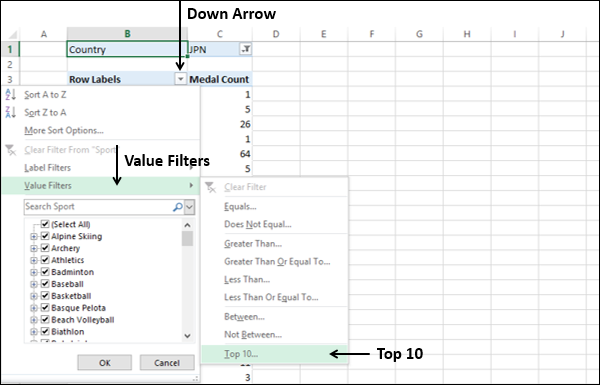Top 10 Filter (<column name>) dialog box appears.

• Under Show, select the following in the boxes from left to right.
• Top
• 18 (The number of top values that you want to display. The default is 10.)
• Items.
• In the by box, select Medal Count.• Click OK. The top 18 values will be displayed in the PivotTable.

• It is simple and easy to use.
• Suitable for tables with large number of rows.

• The filter is solely for display purposes.

• If the data underlying the PivotTable changes, you must manually refresh the PivotTable to see the changes.

## Creating a DAX Formula That Dynamically Ranks Values

You can create a calculated column using a DAX formula that contains the ranked values. You can then use a slicer on the resulting calculated column to select the values to be displayed.

You can obtain a rank value for a given value in a row by counting the number of rows in the same table having a value larger than the one that is being compared. This method returns the following −

• A zero value for the highest value in the table.

• Equal values will have the same rank value. If n number of values are equal, the next value after the equal values will have a nonconsecutive rank value adding up the number n.

For example, if you have a table ‘Sales’ with sales data, you can create a calculated column with the ranks of the Sales Amount values as follows −

```= COUNTROWS (FILTER (Sales,
EARLIER (Sales [Sales Amount]) < Sales [Sales Amount])
) + 1
```

Next, you can insert a Slicer on the new calculated column and selectively display the values by ranks.

• The ranking is done in the table and not on a PivotTable. Hence, can be used in any number of PivotTables.

• DAX formulas are calculated dynamically. Hence, you can always be sure that the ranking is correct even if the underlying data has changed.

• Since the DAX formula is used in a calculated column, you can use the ranking in a Slicer.

• Suitable for tables with large number of rows.